# Concise Selina Solutions for Class 9 Maths Chapter 23 - Trigonometrical Ratios of Standard Angles

Selina Solutions are considered to be very useful when you are preparing for the ICSE Class 9 Maths exams. Here, we bring to you the Selina Solutions for Class 9 Maths, providing the detailed explanations to the questions present in the exercises of Chapter 23- Trigonometrical Ratios of Standard Angles. These answers have been devised by the subject matter experts as per the syllabus prescribed by the CISCE for the ICSE.

Here we have provided the complete solutions of Class 9 Maths Chapter 23- Trigonometrical Ratios of Standard Angles in PDF format. You can avail these Selina solutions and download it for free to practice them offline as well.

### Download PDF of Selina Solutions for Class 9 Maths Chapter 23- Trigonometrical Ratios of Standard Angles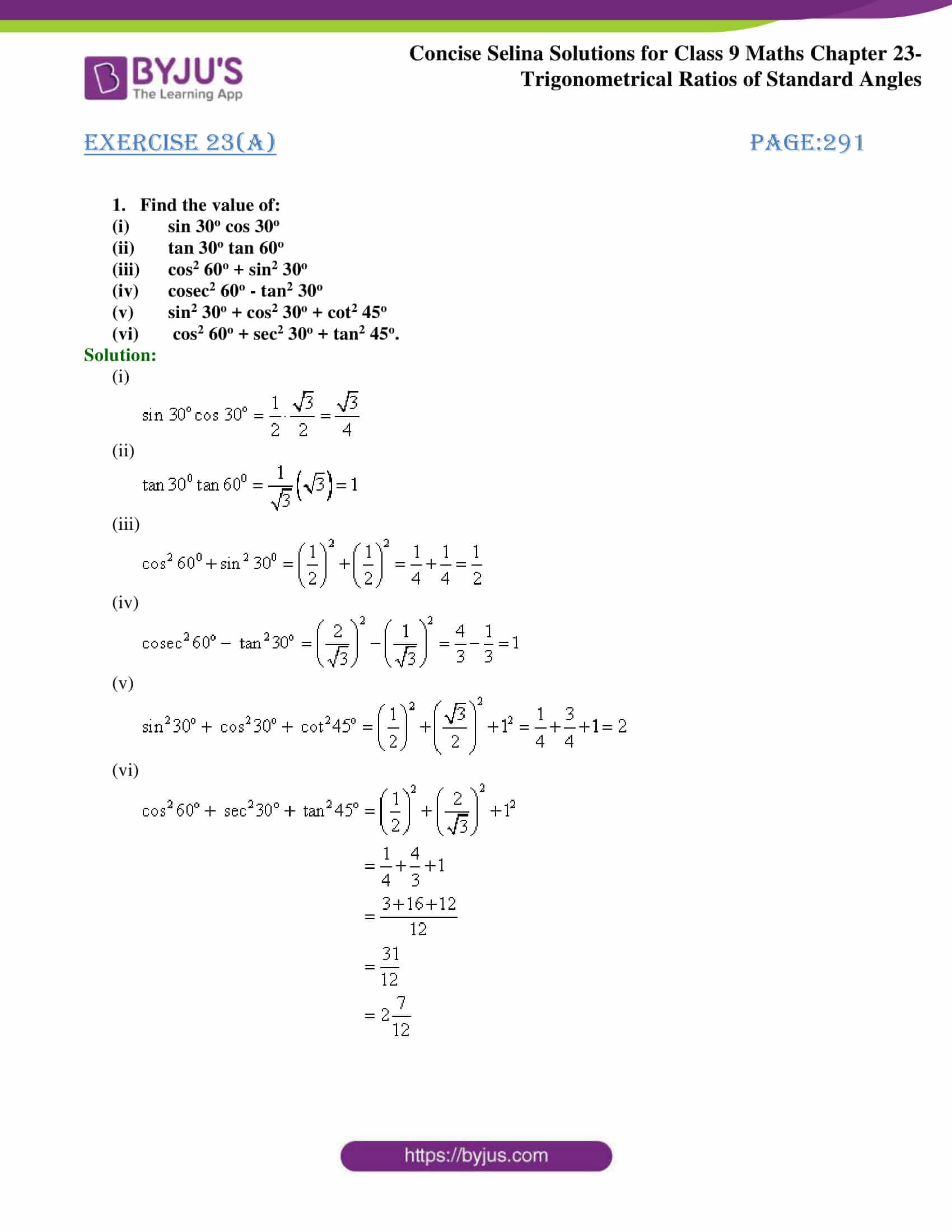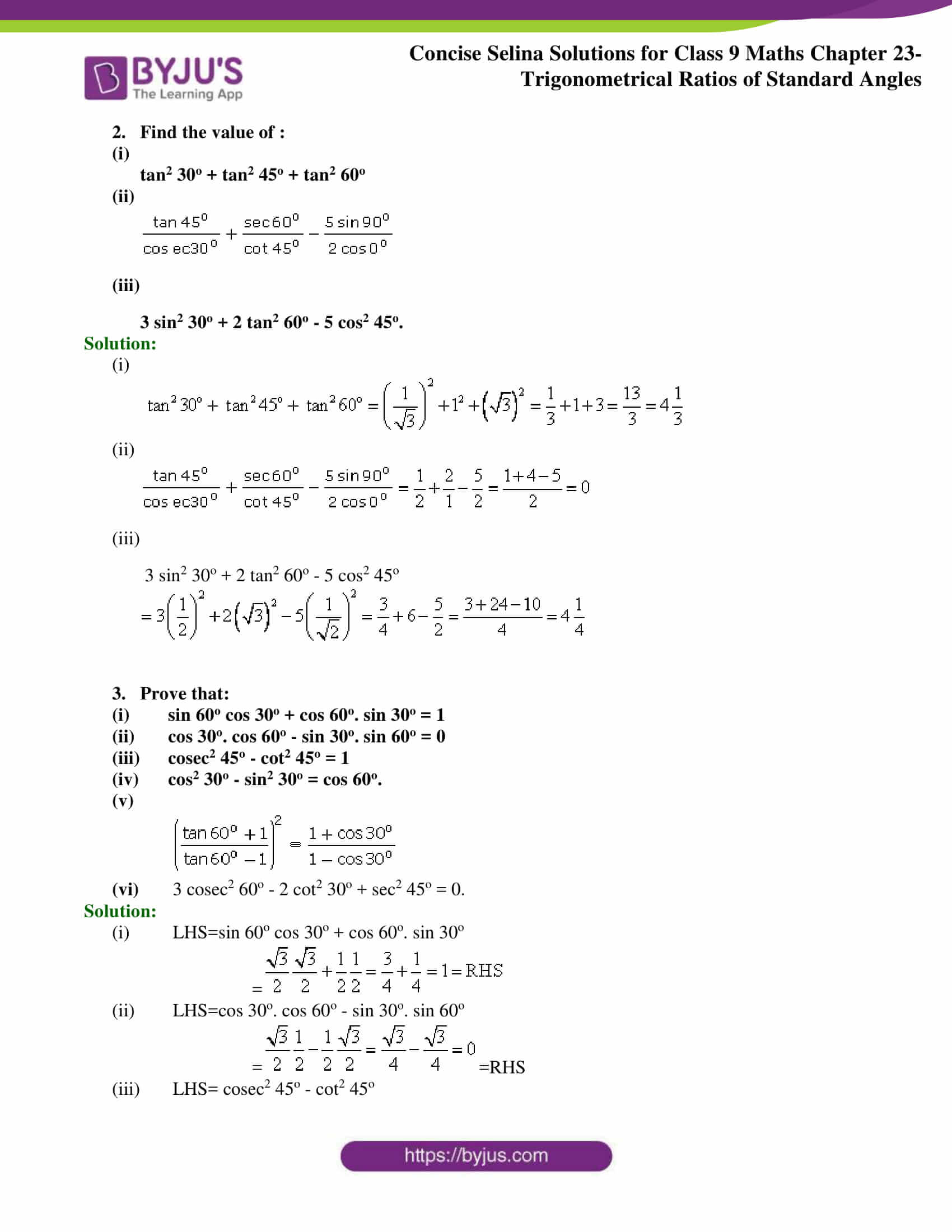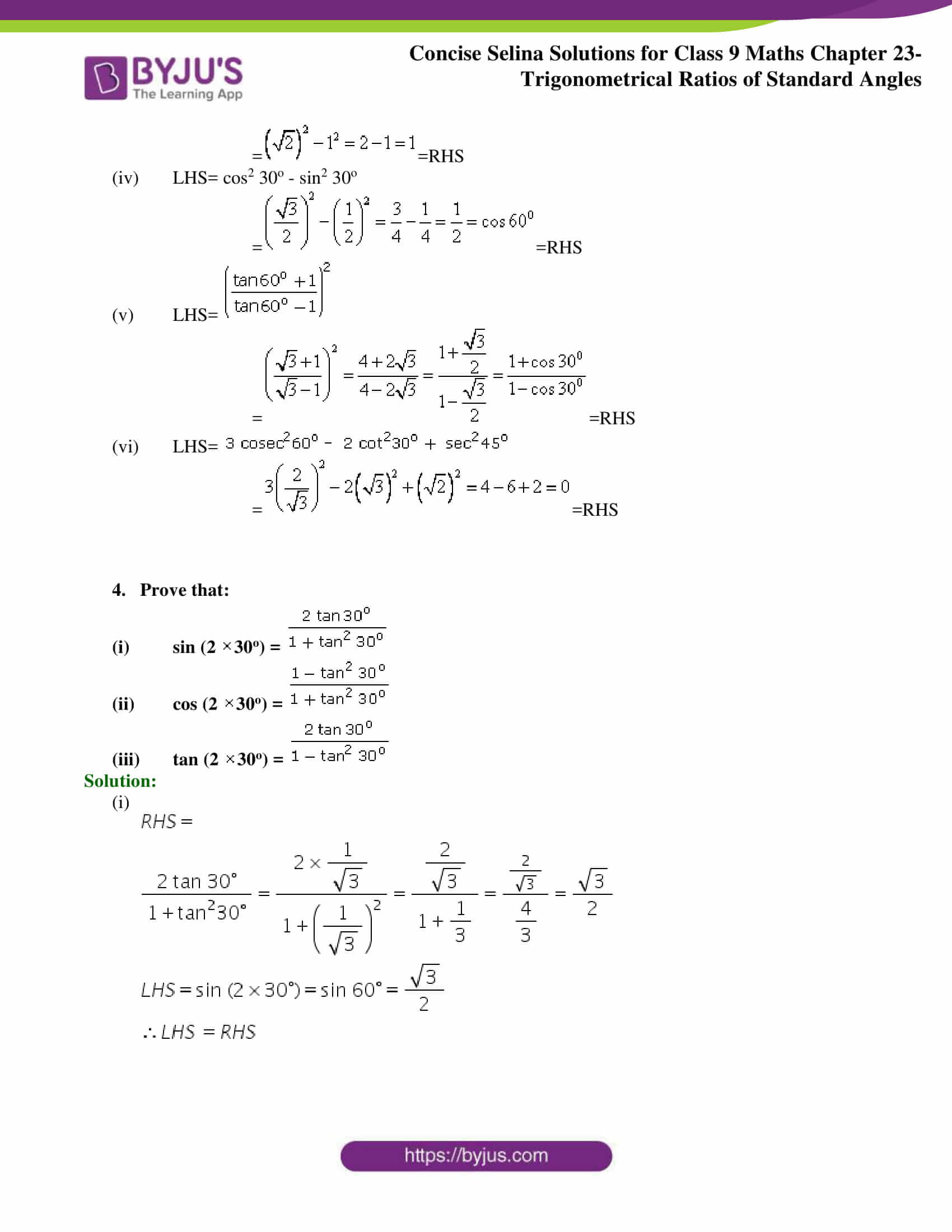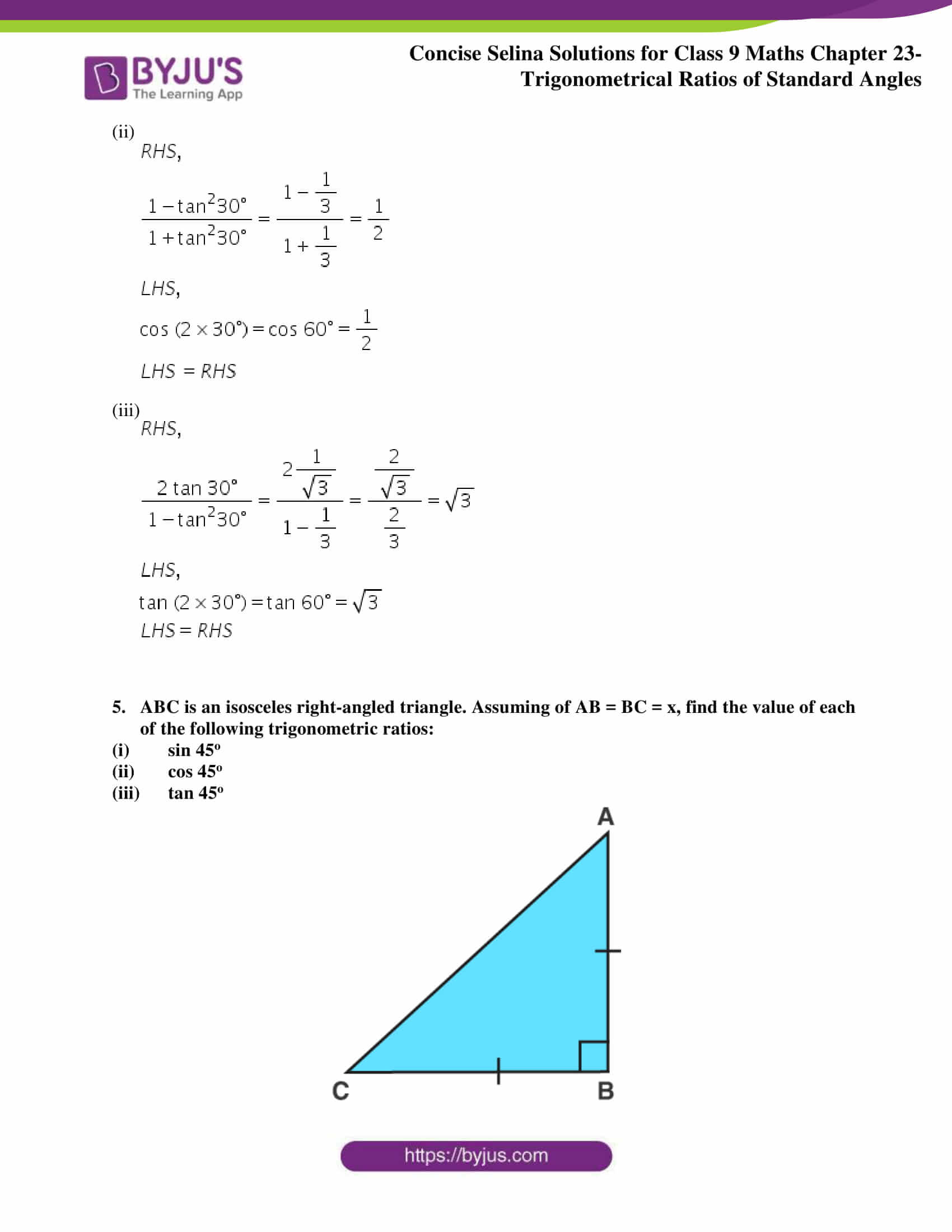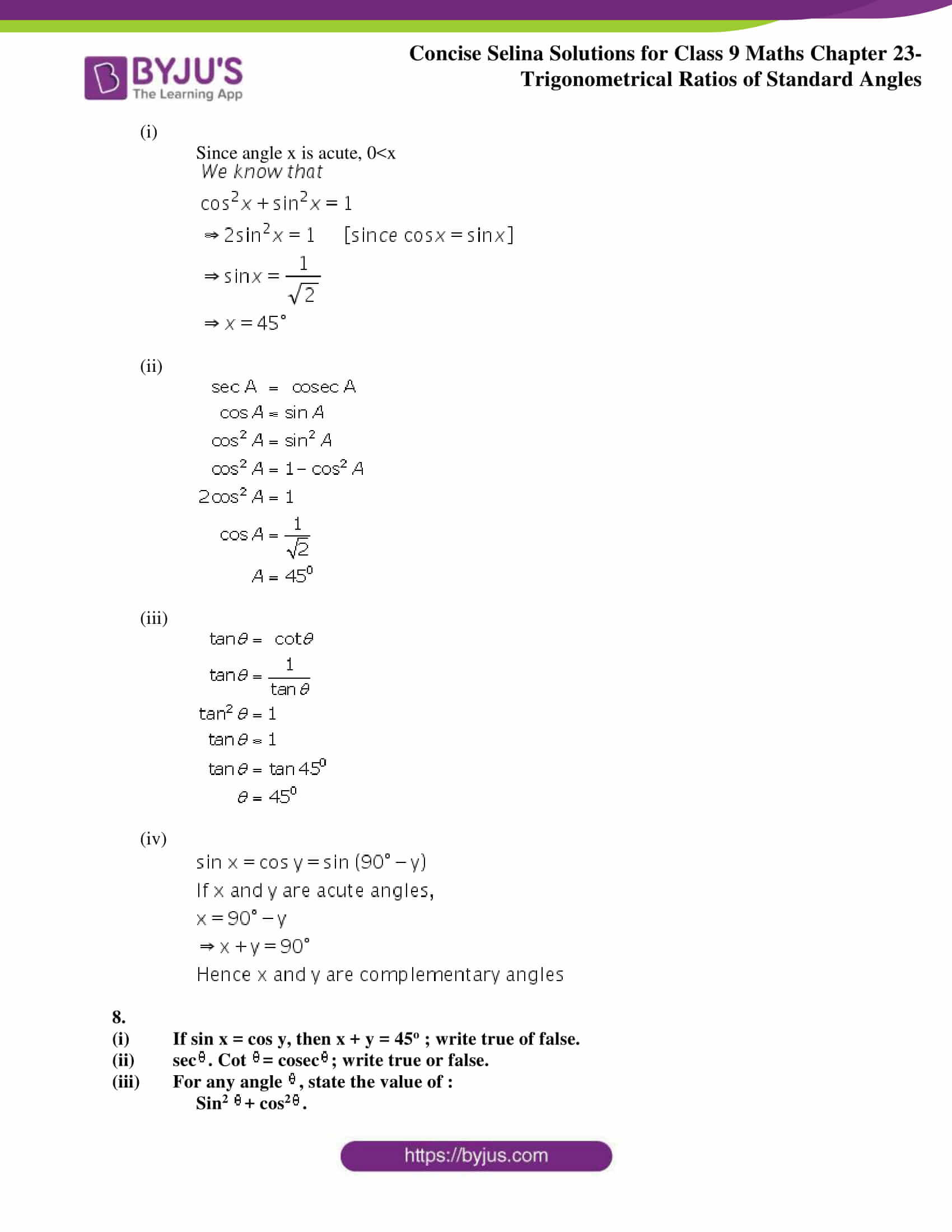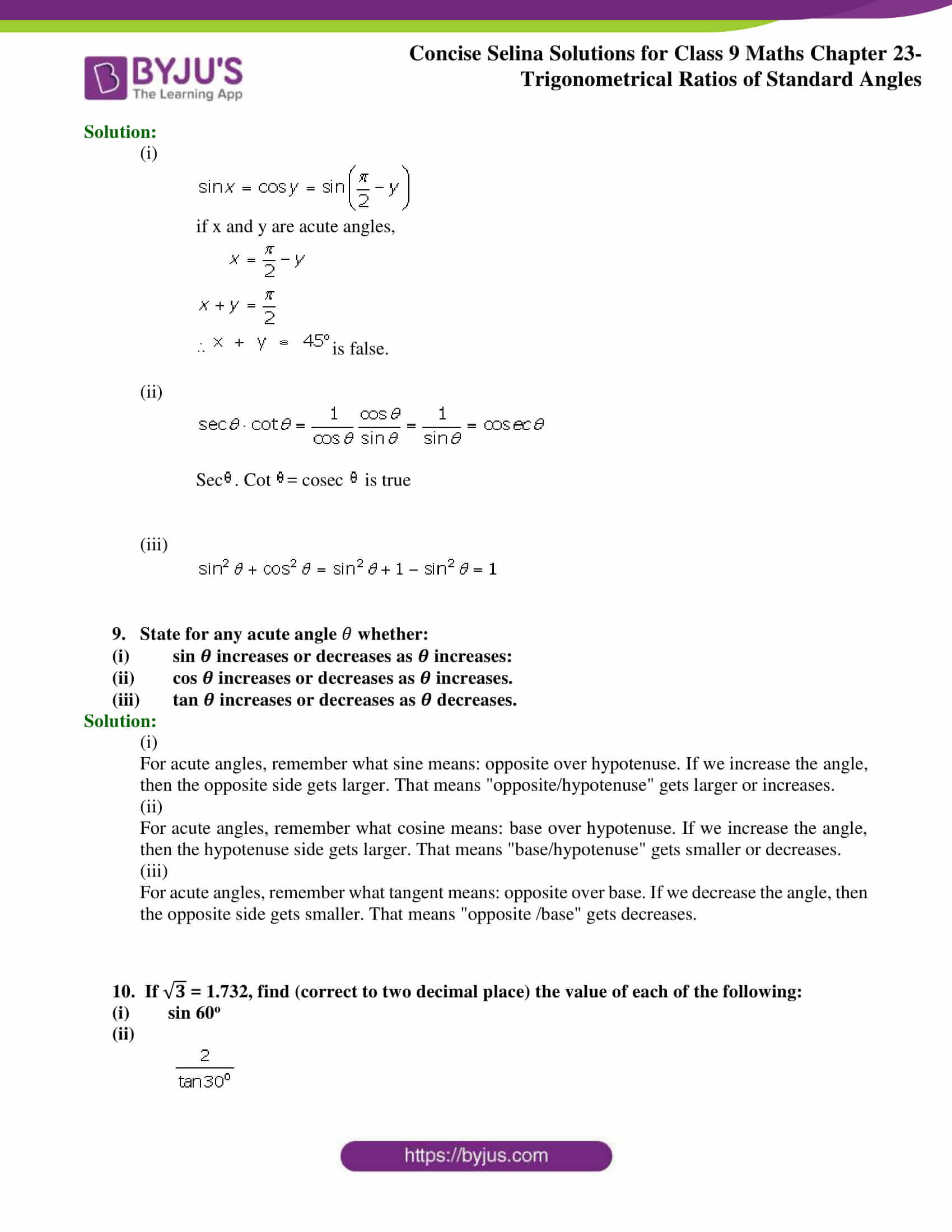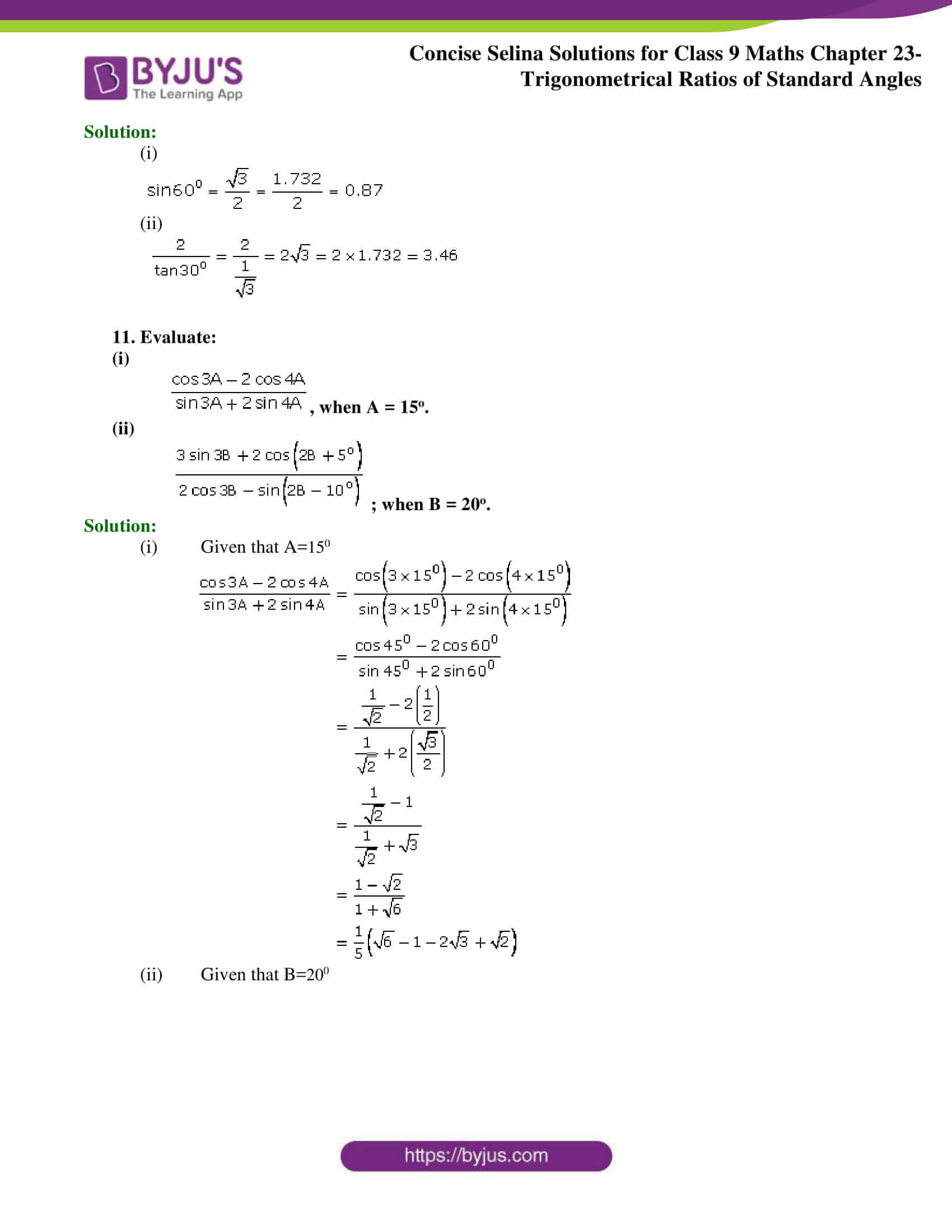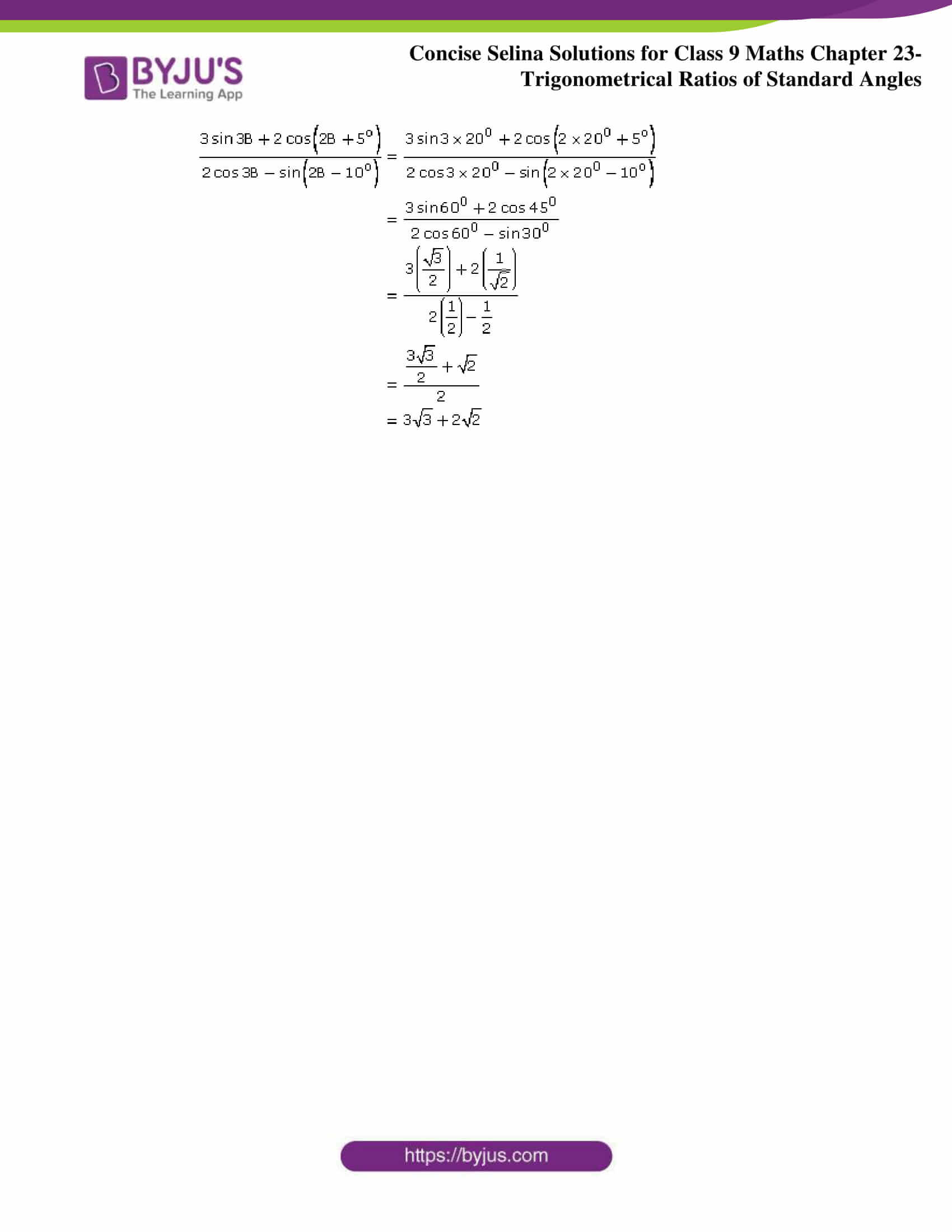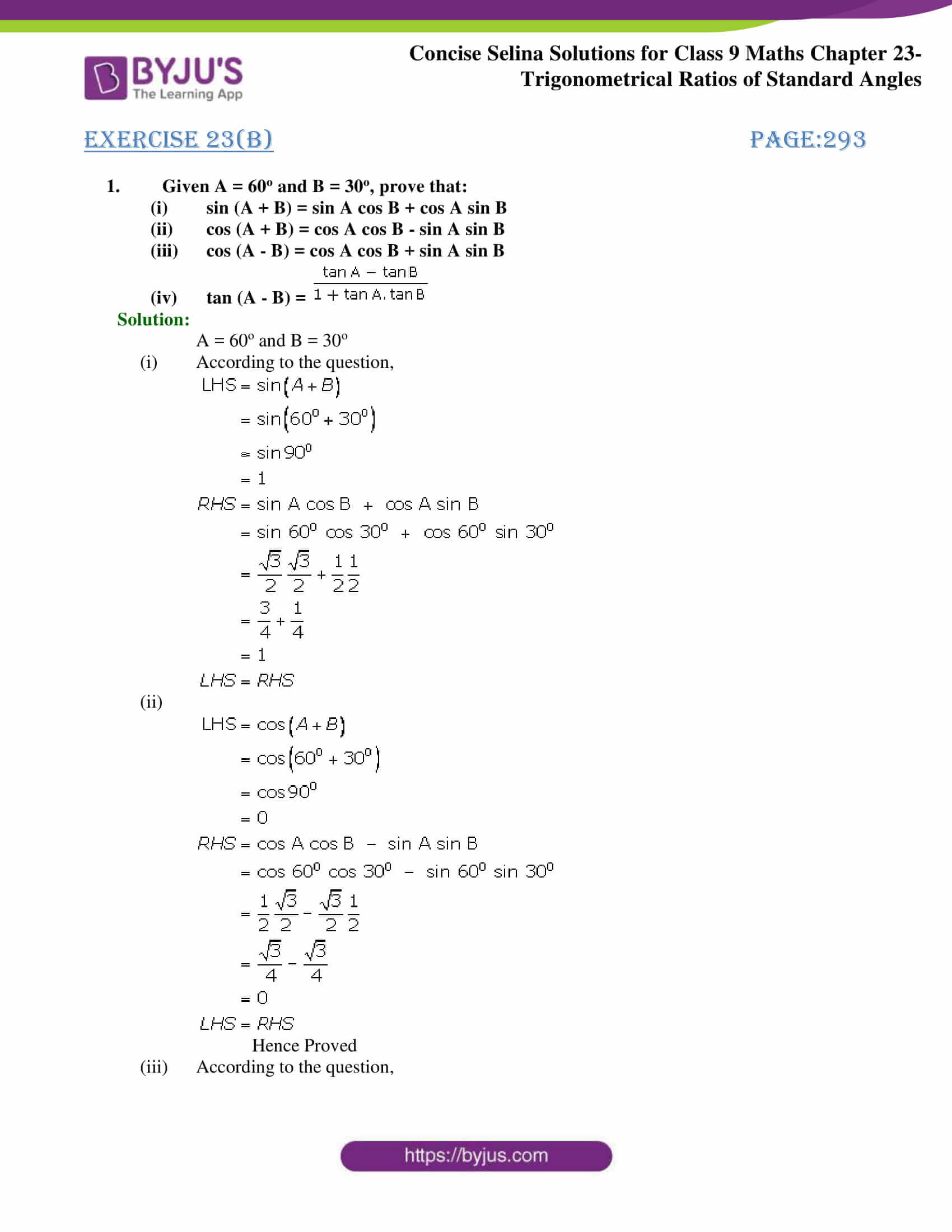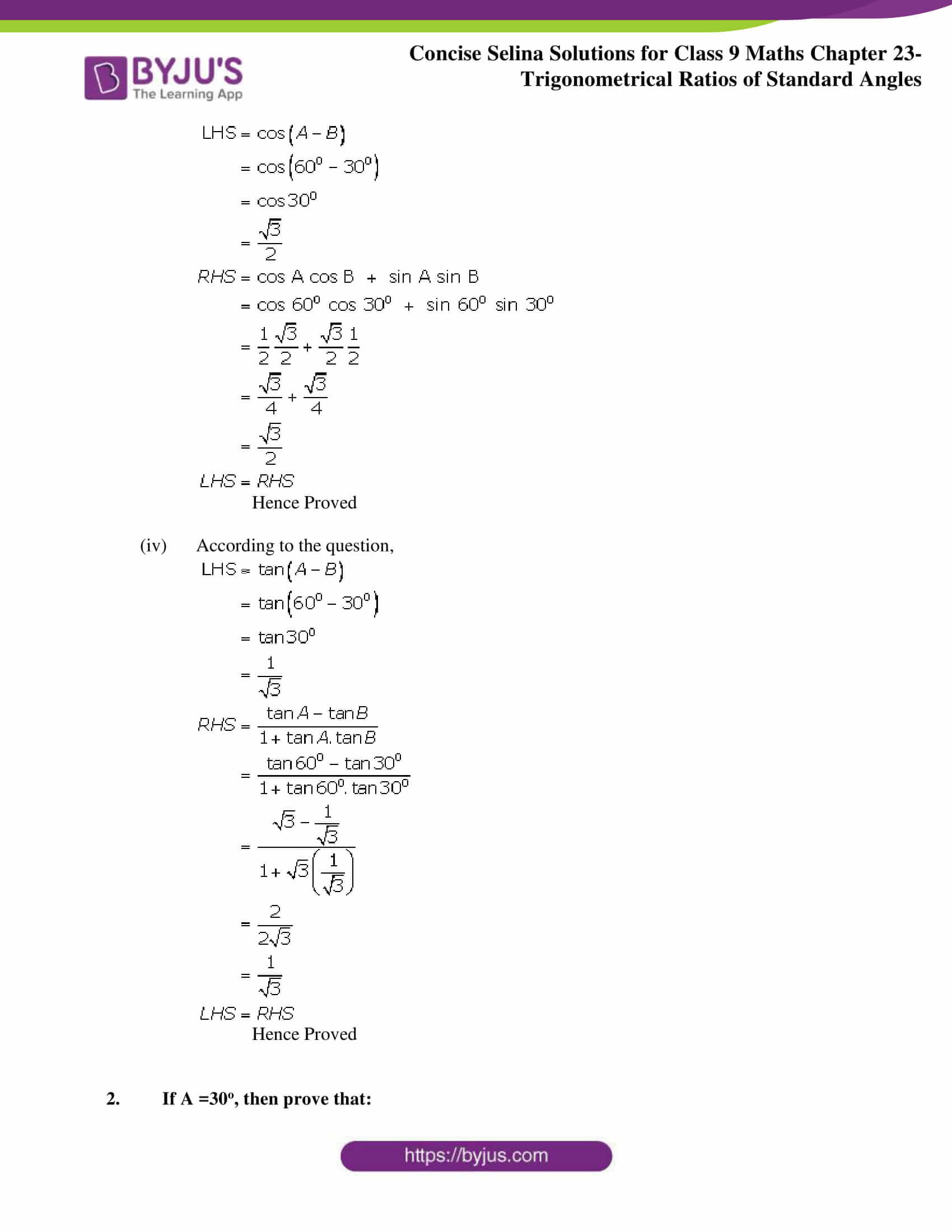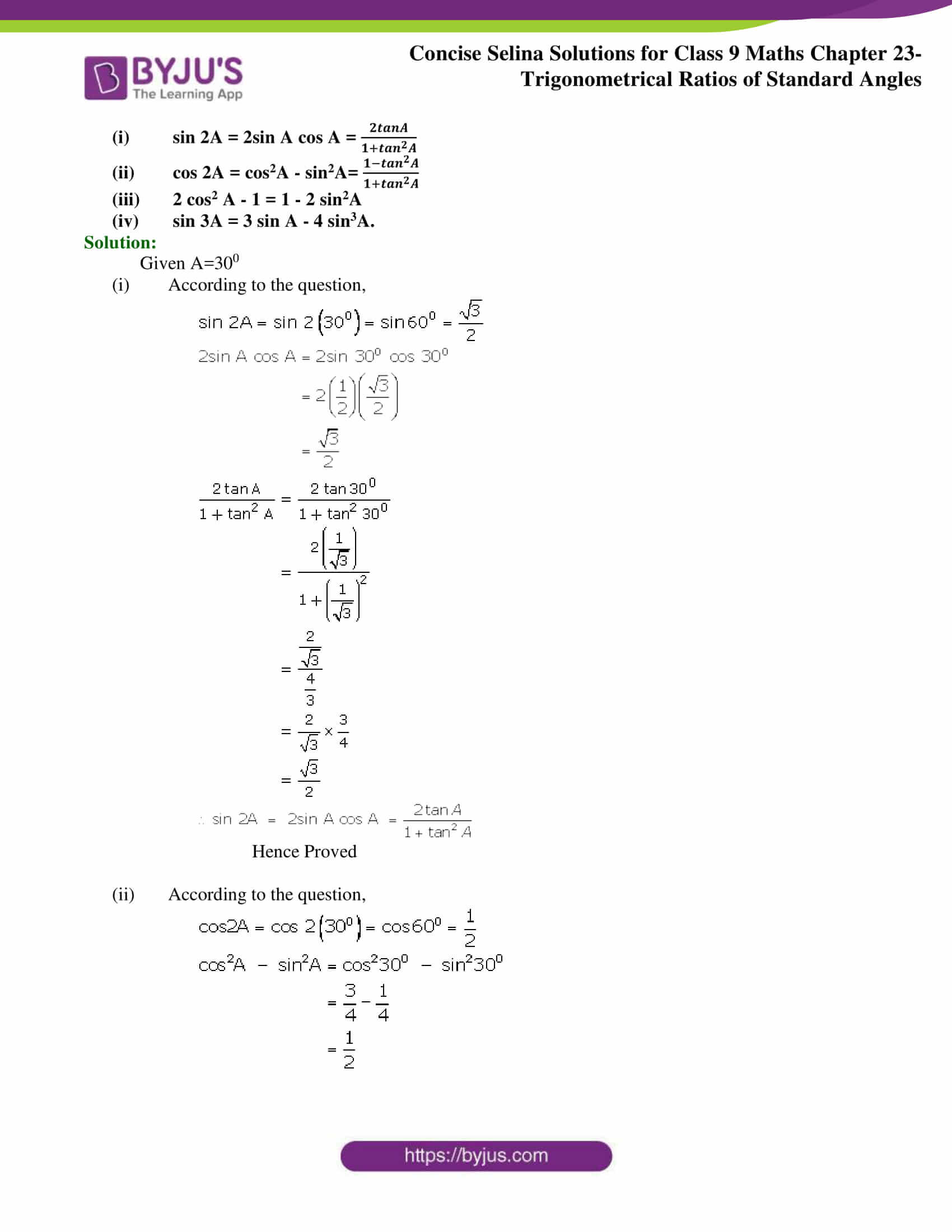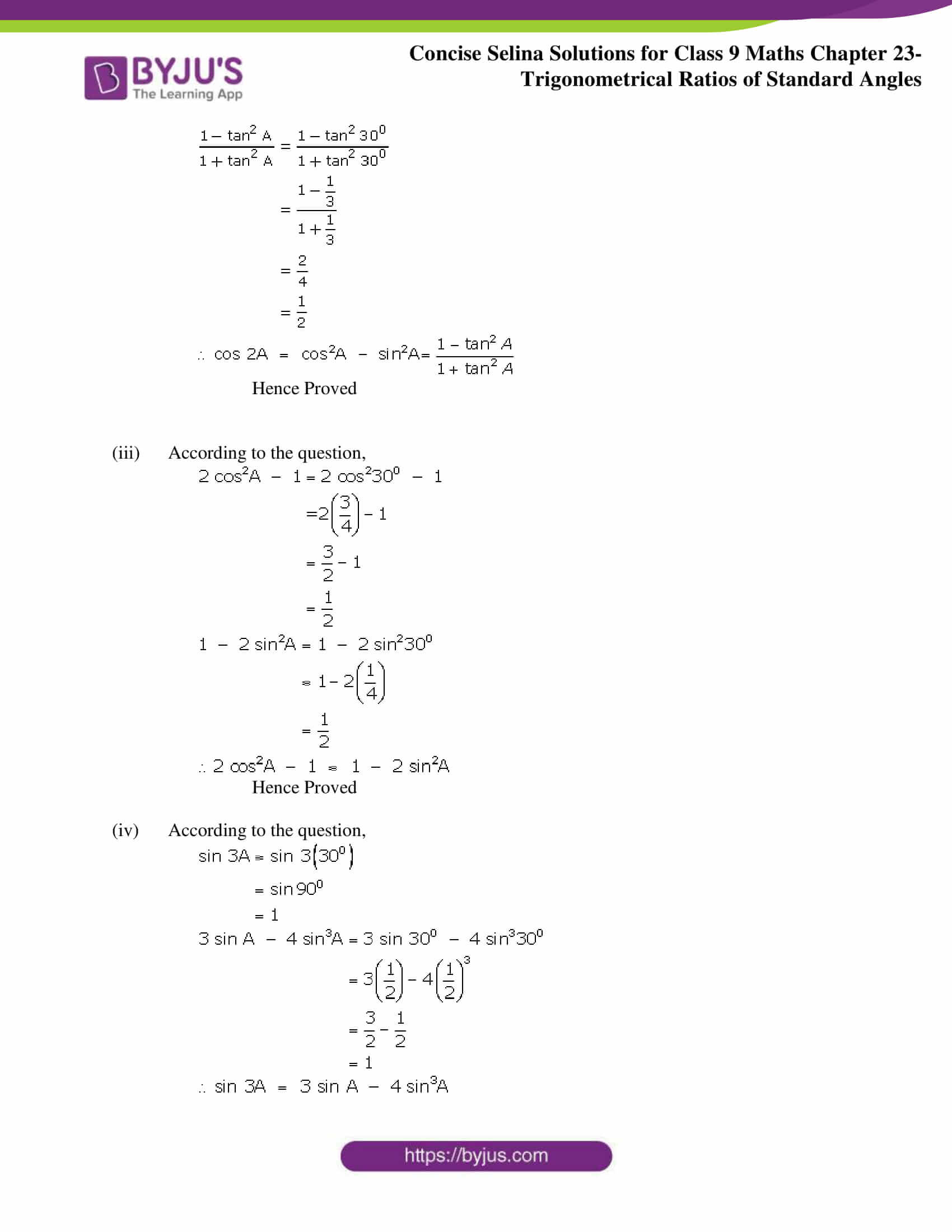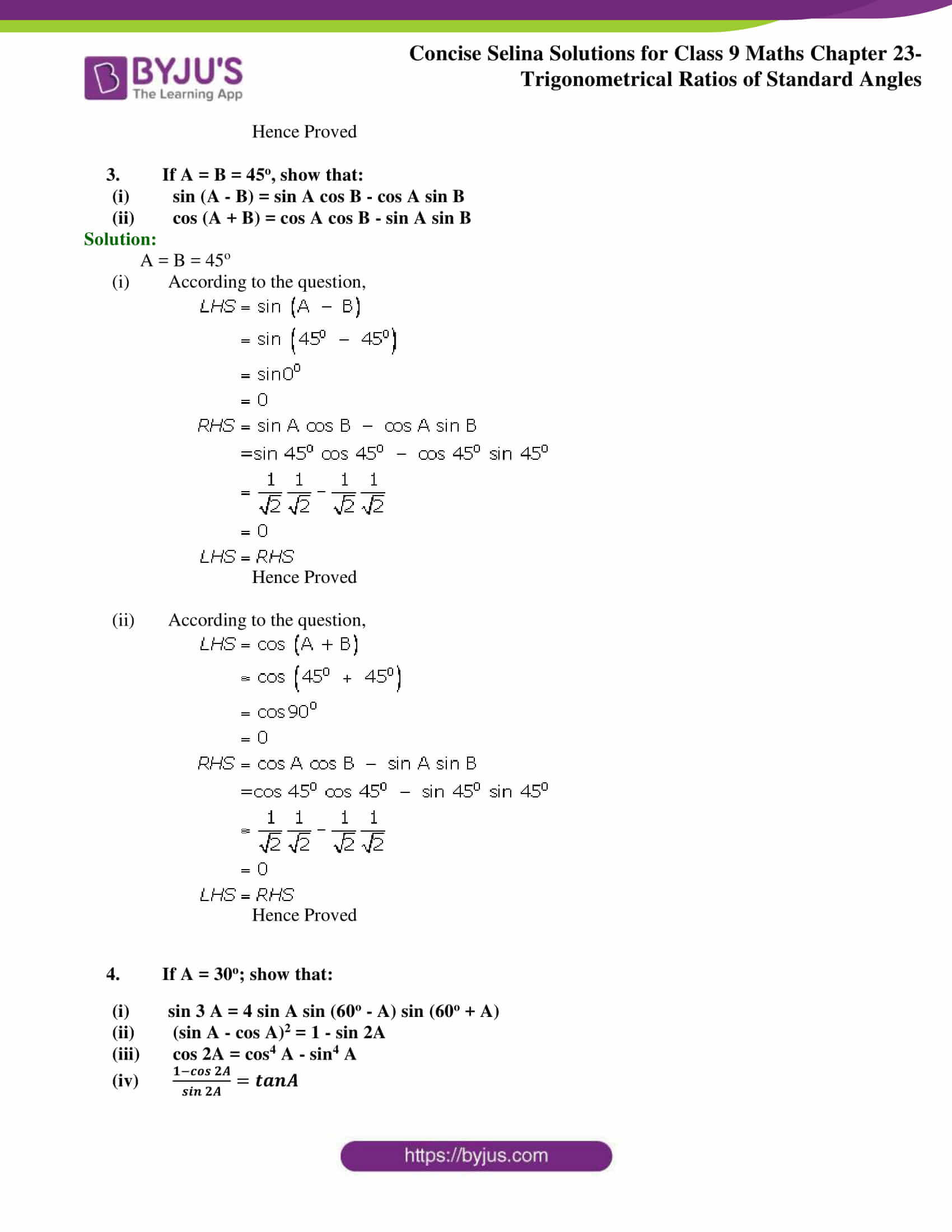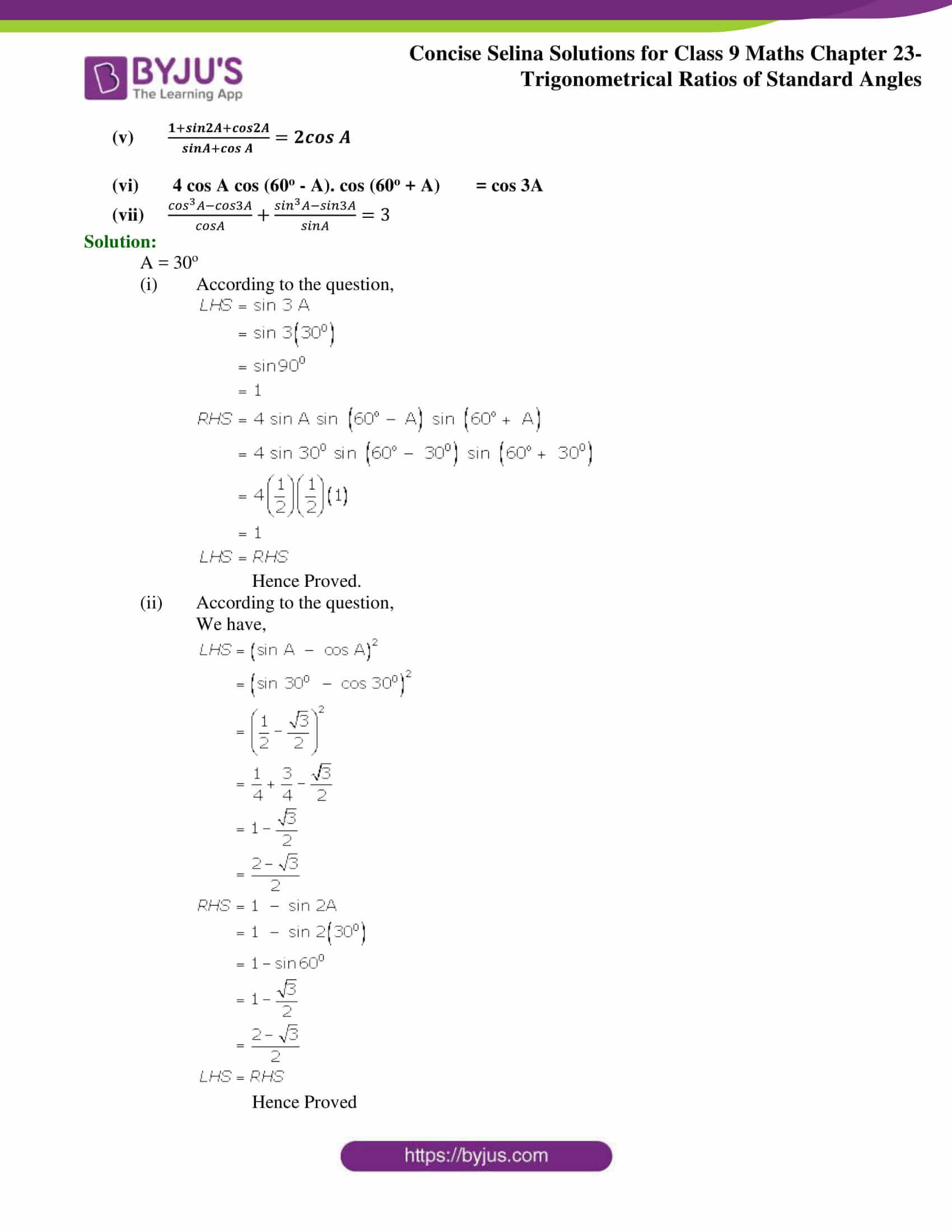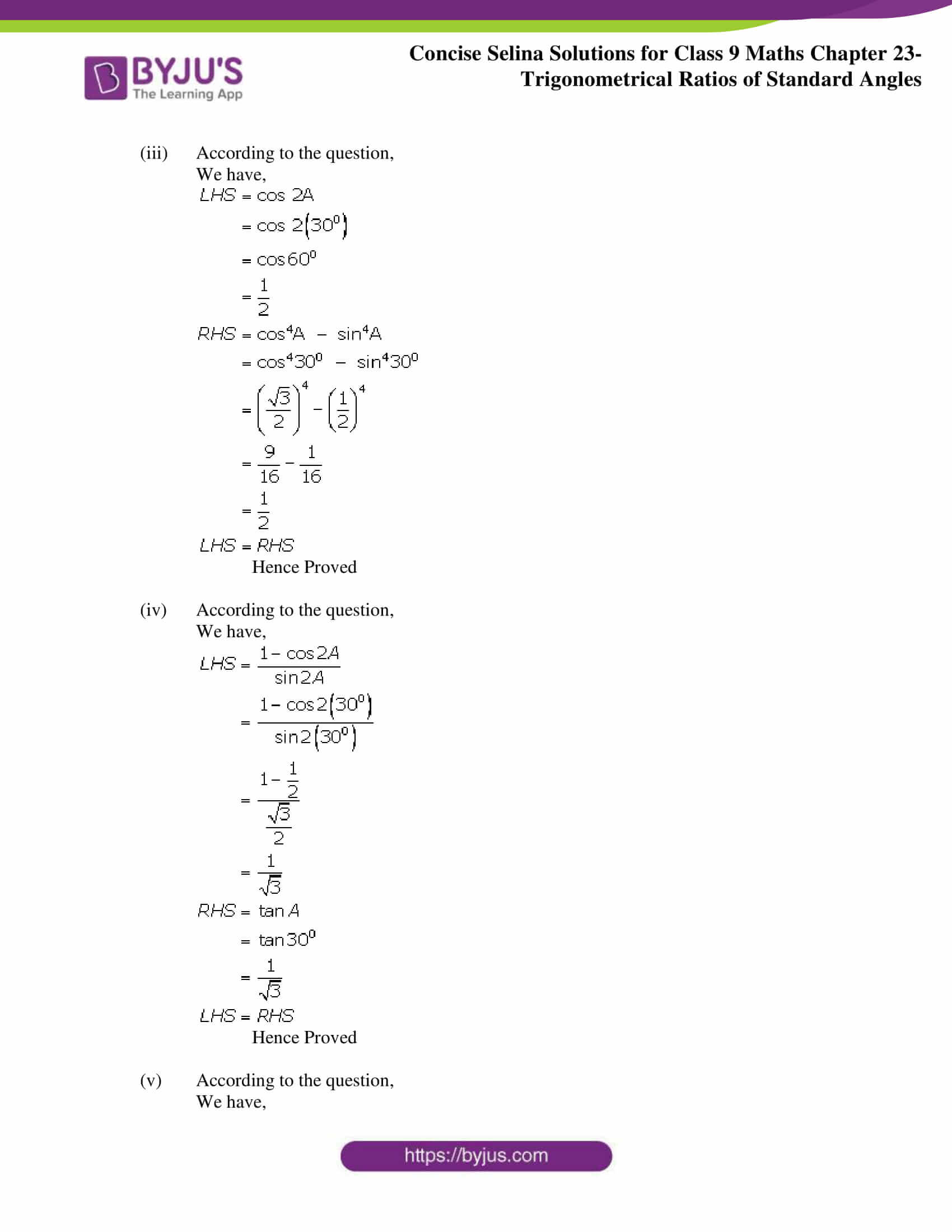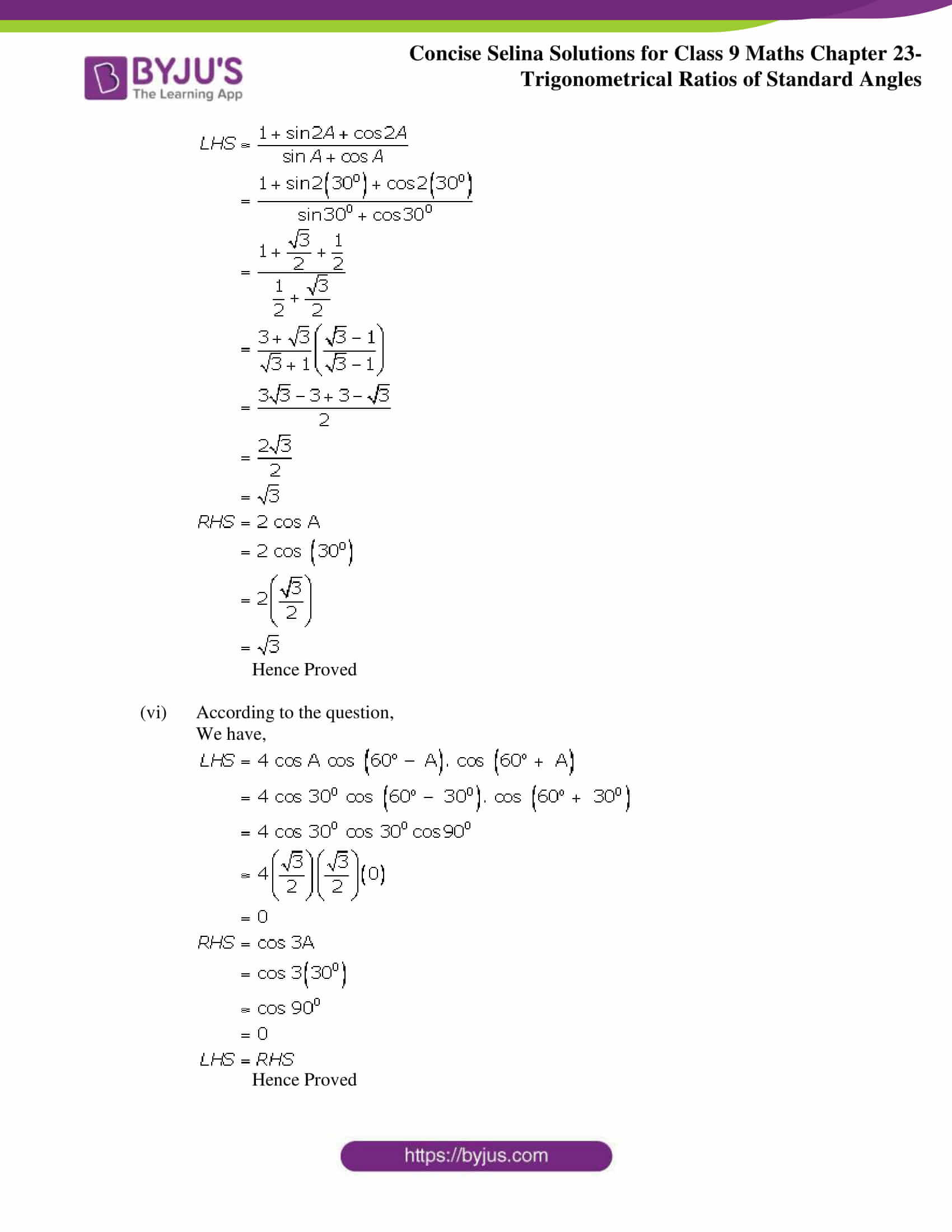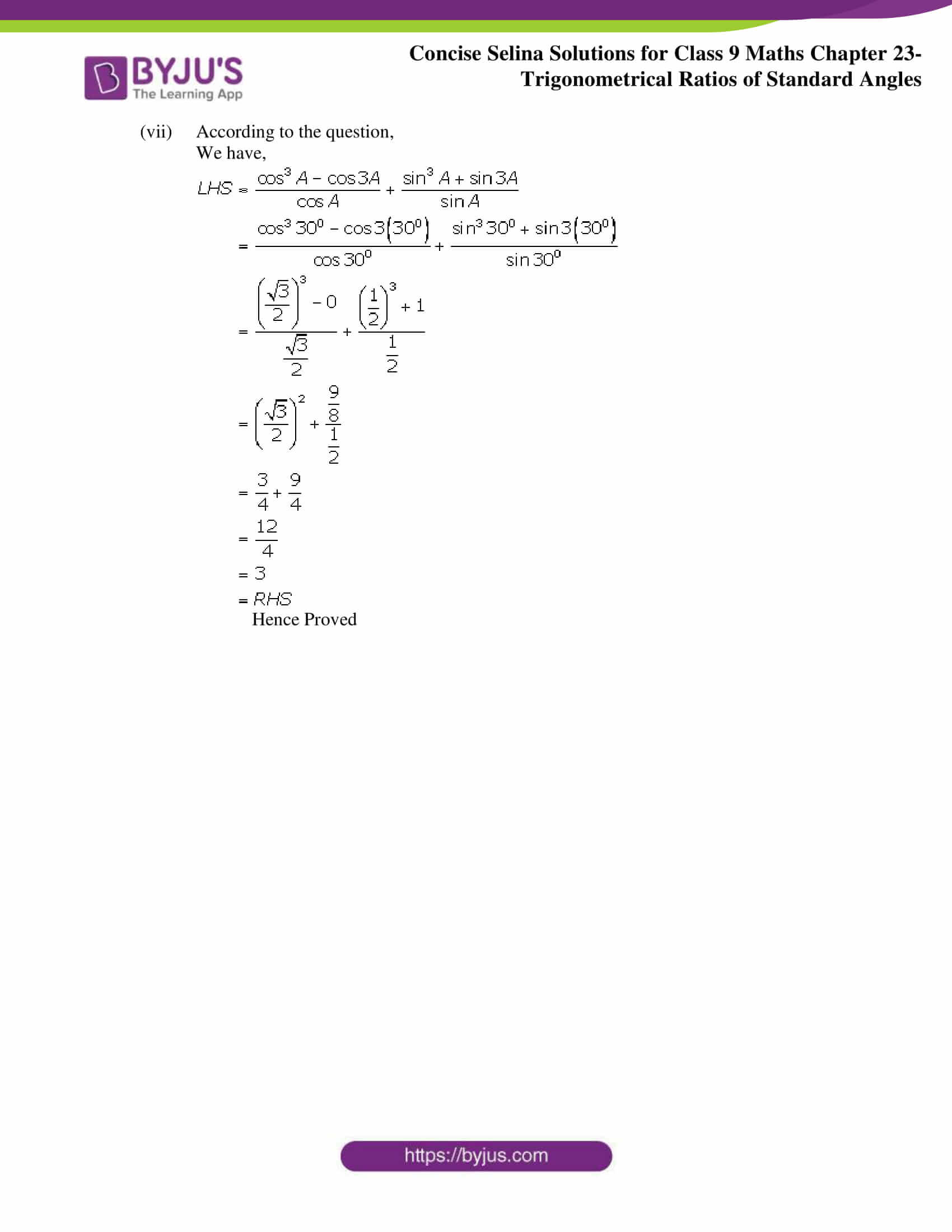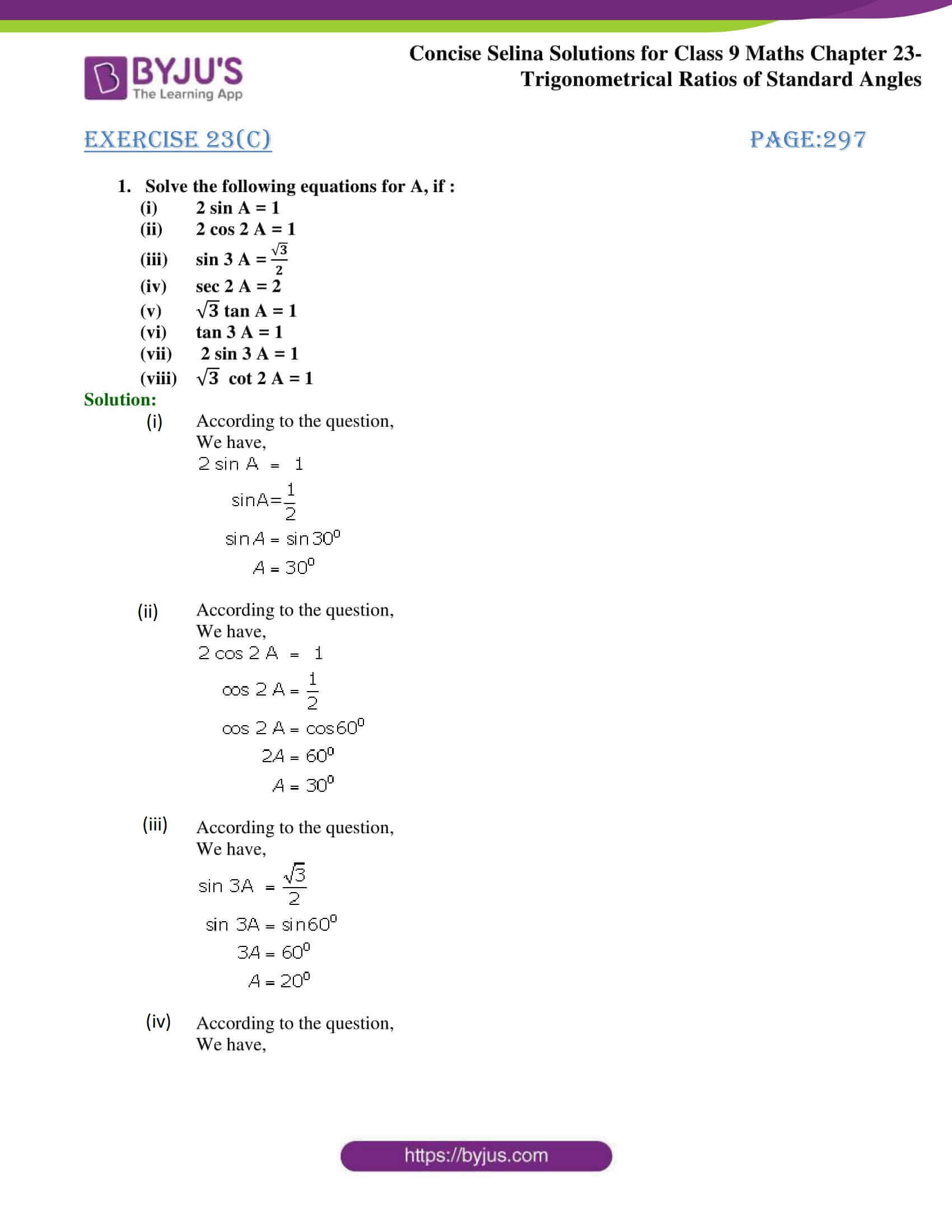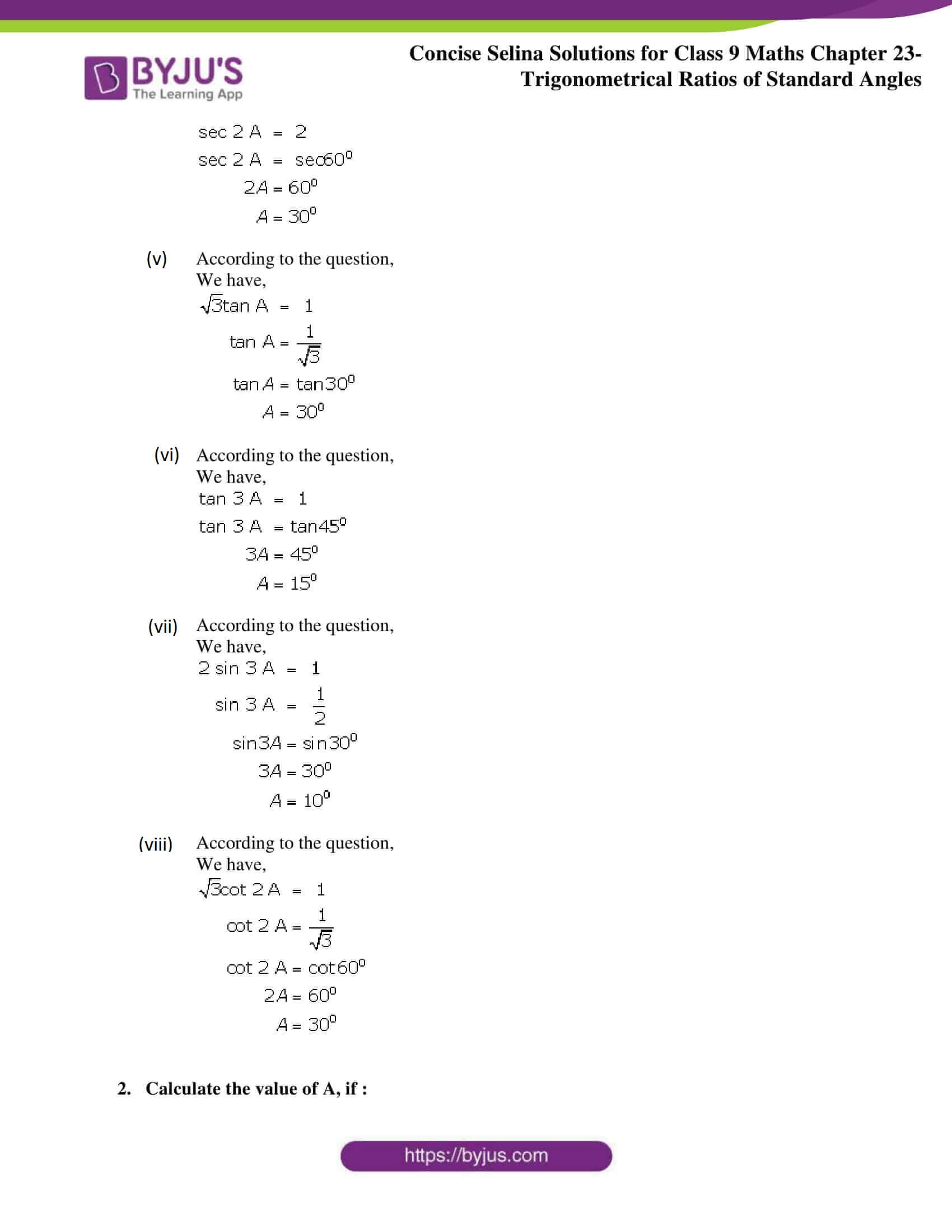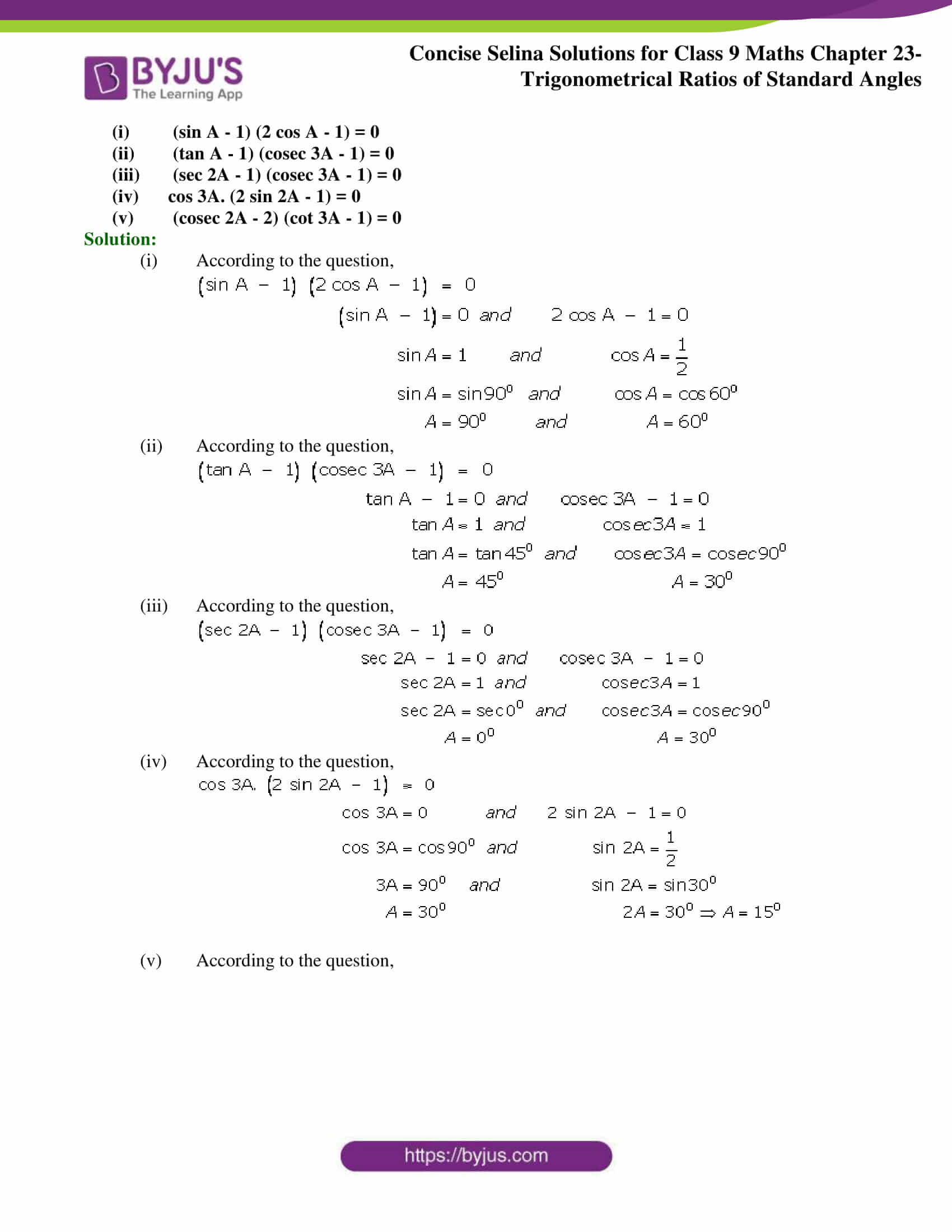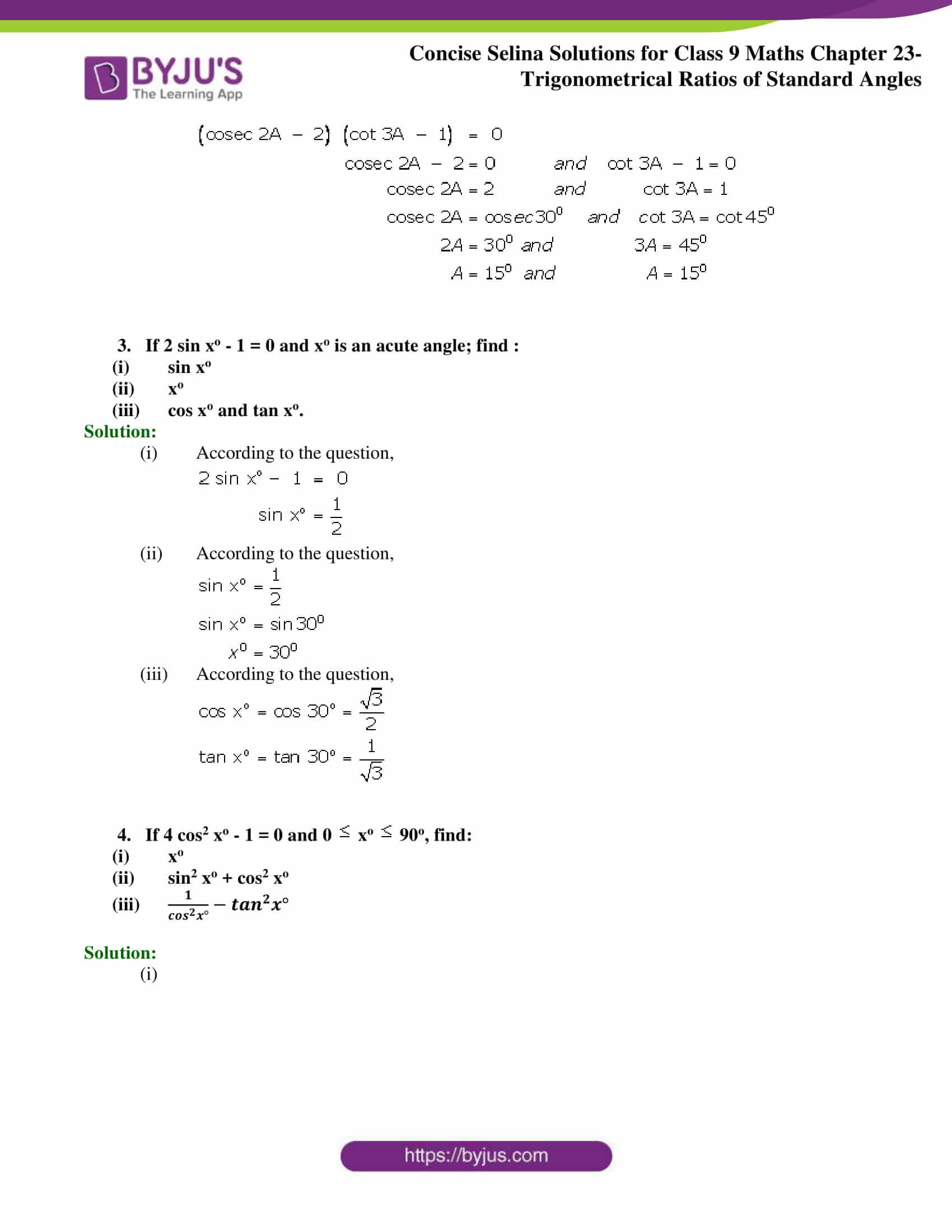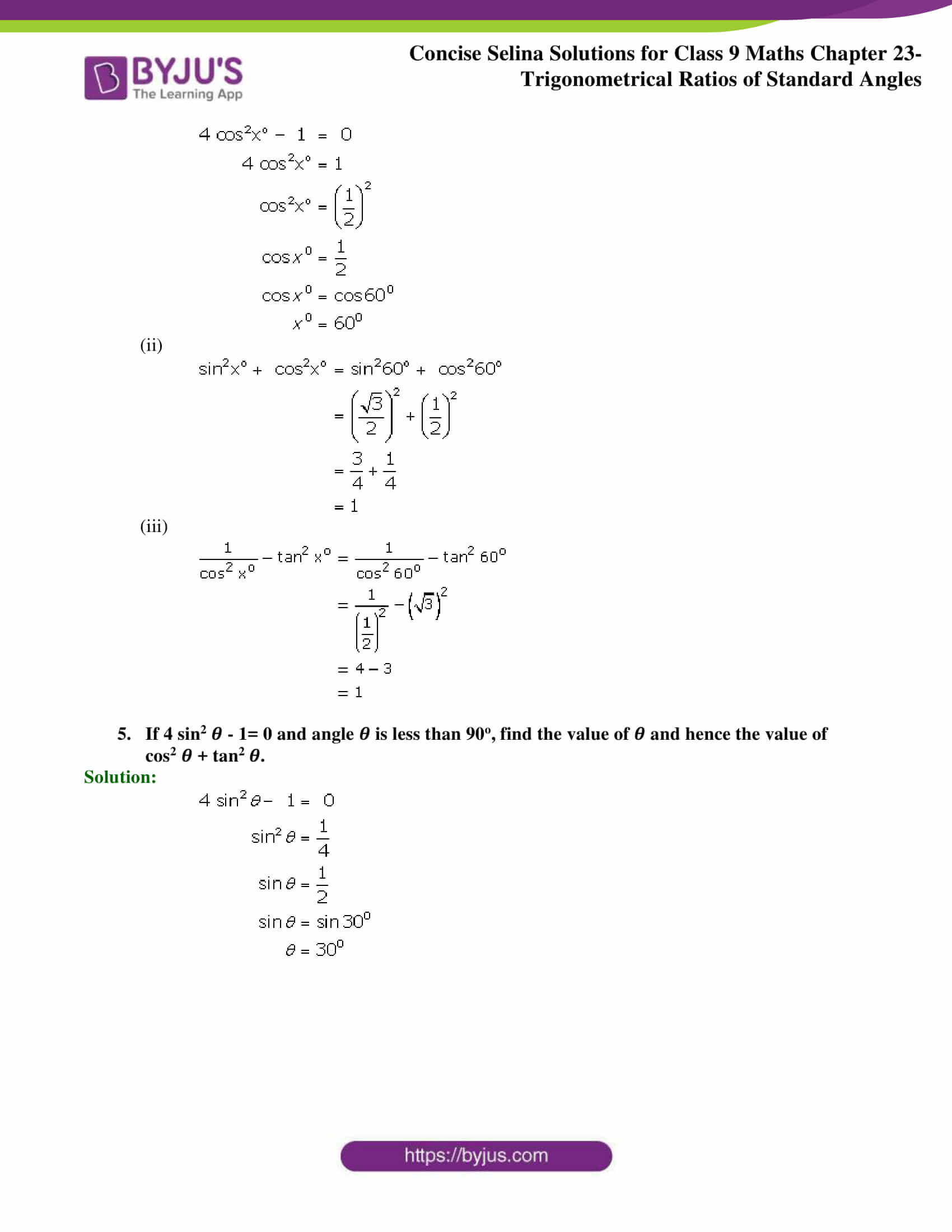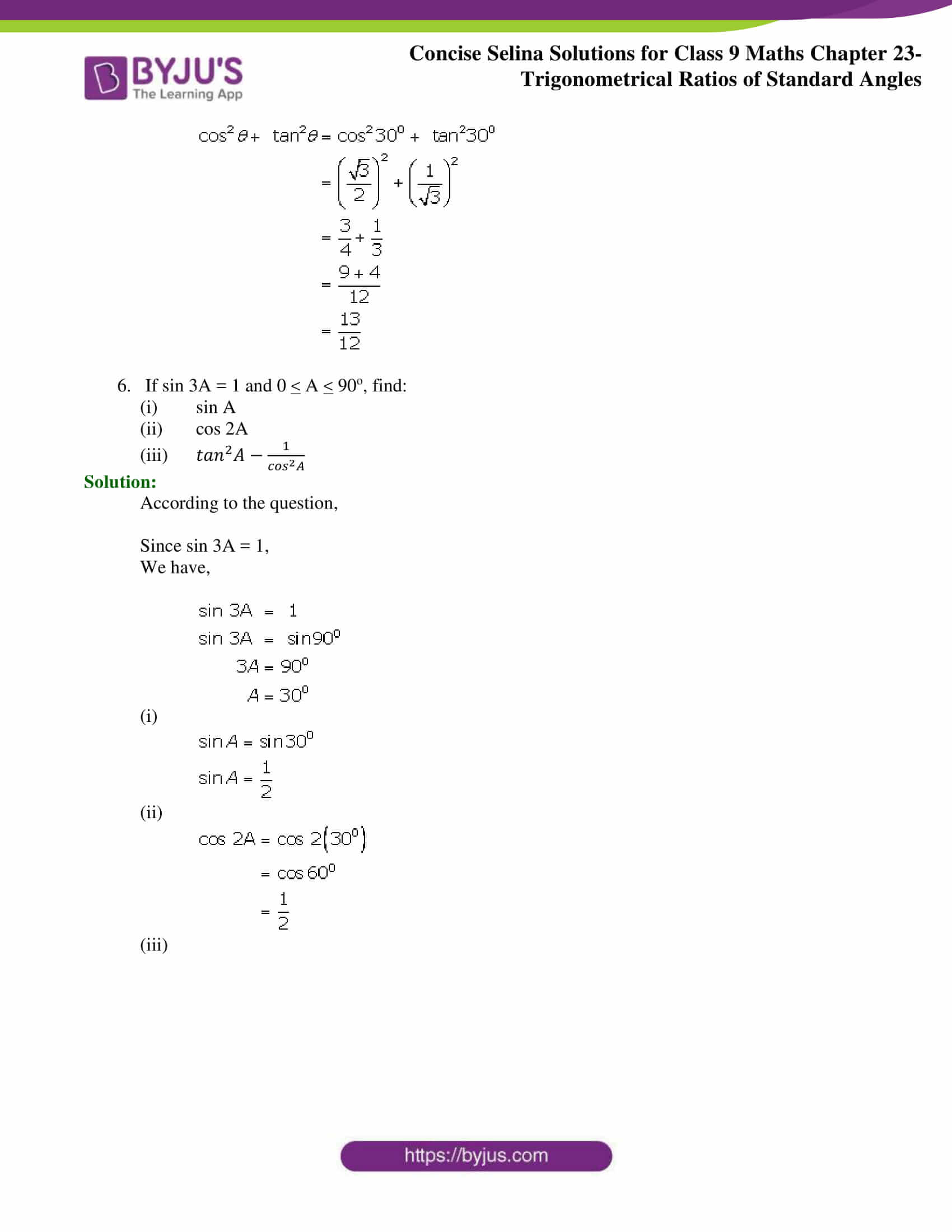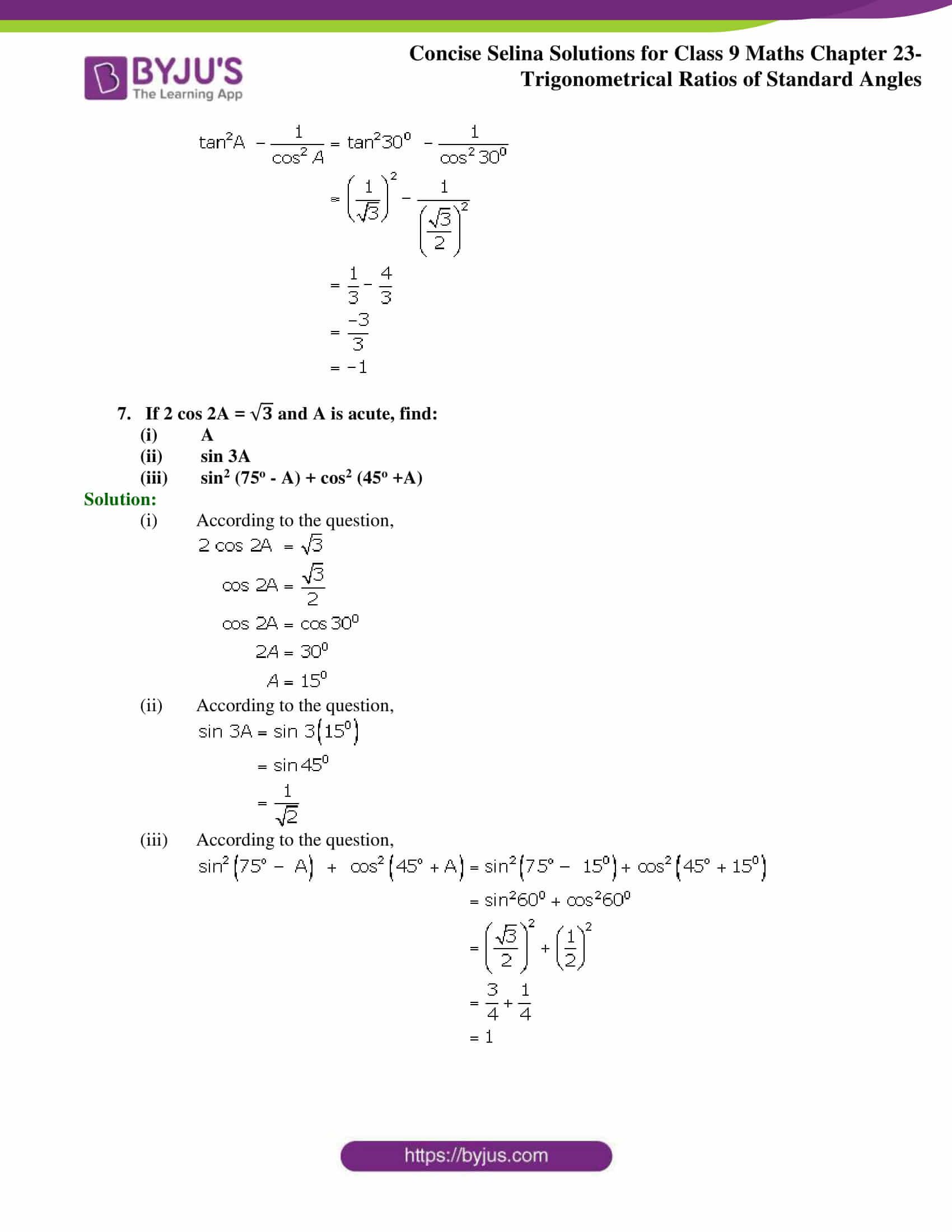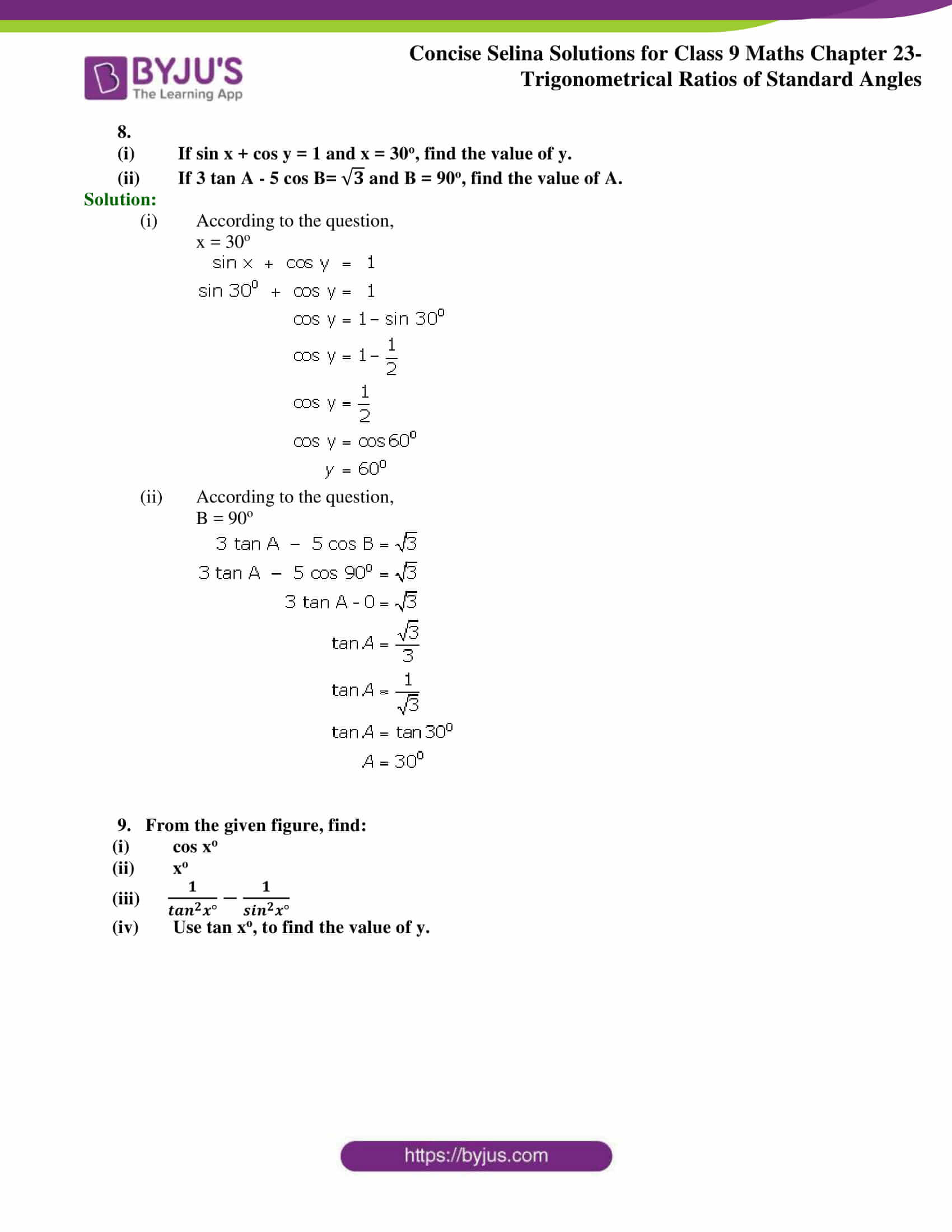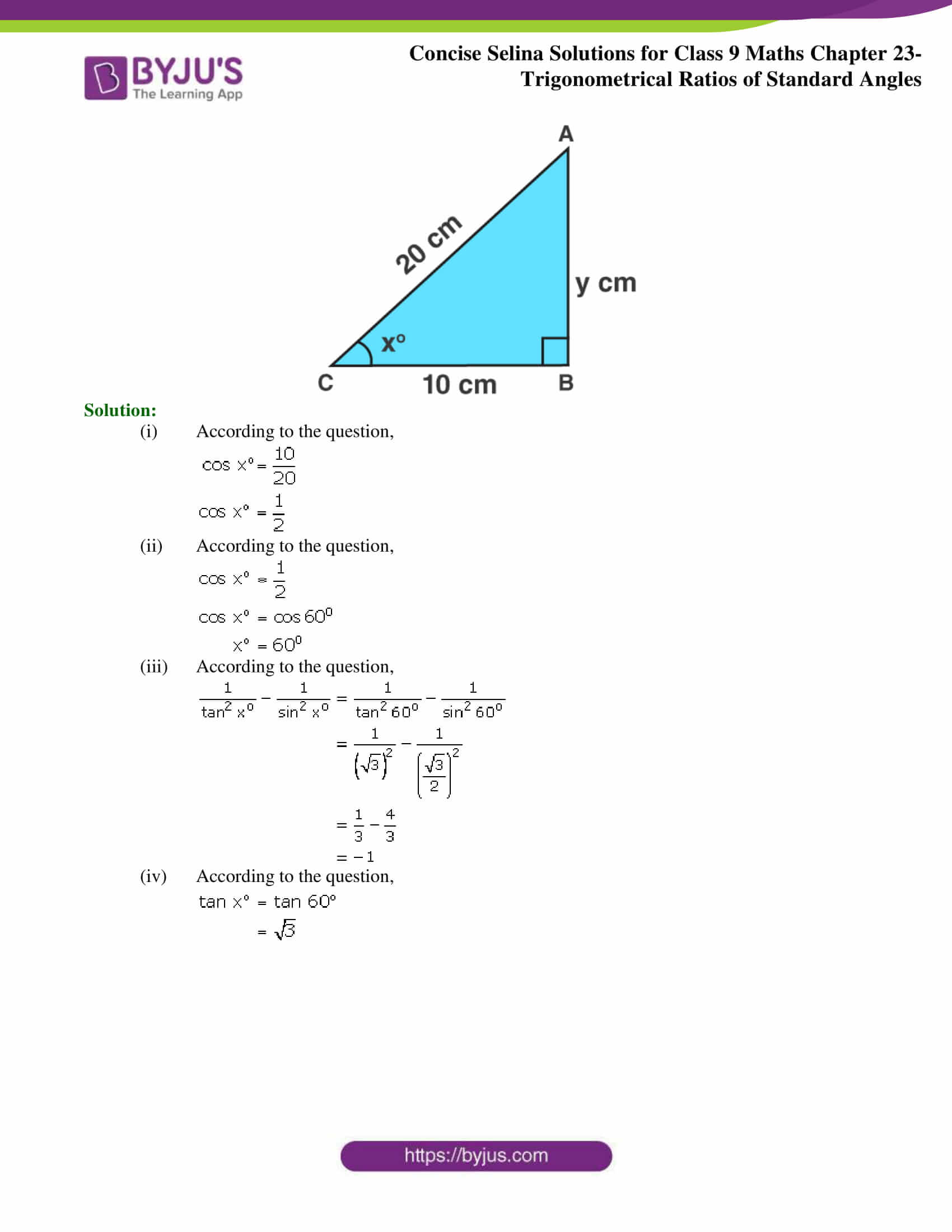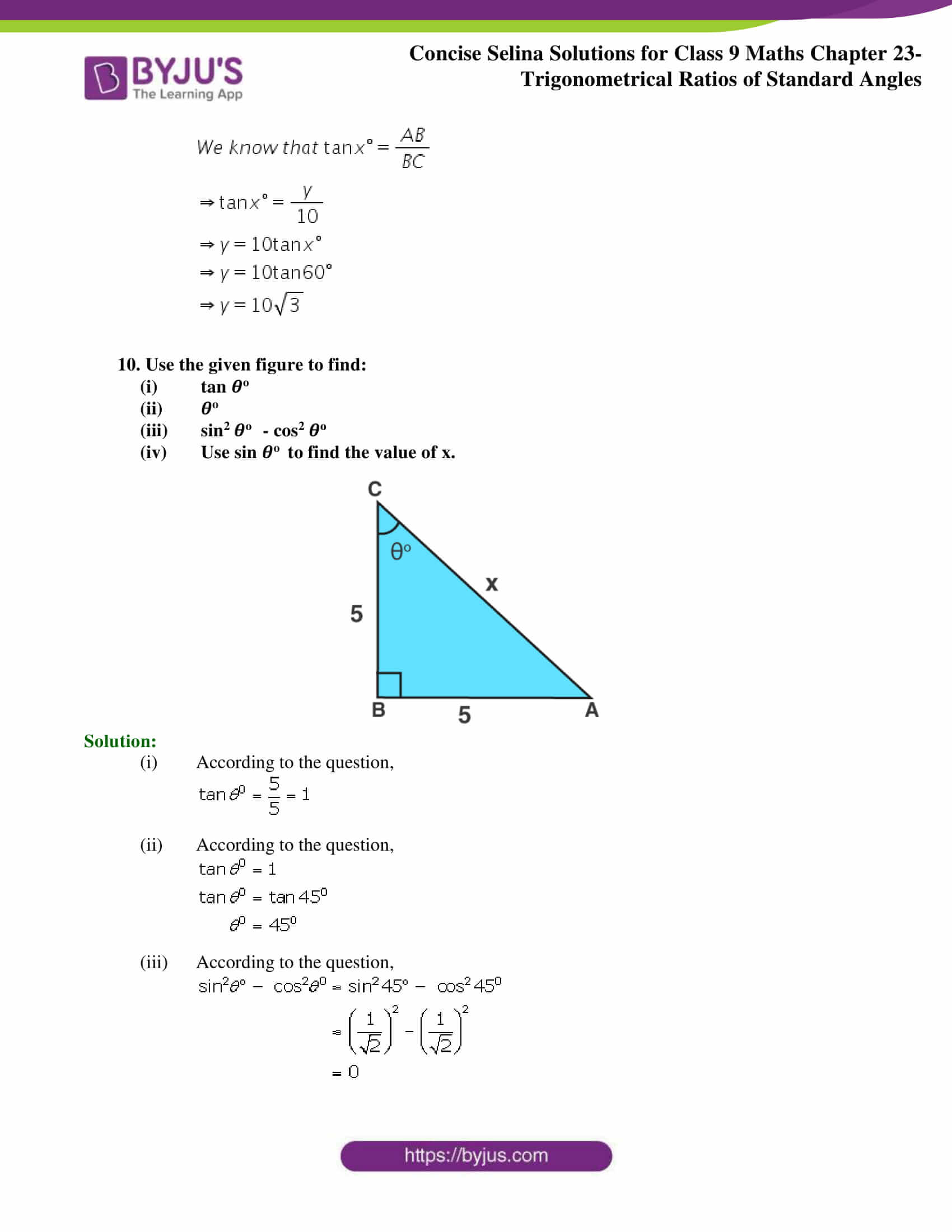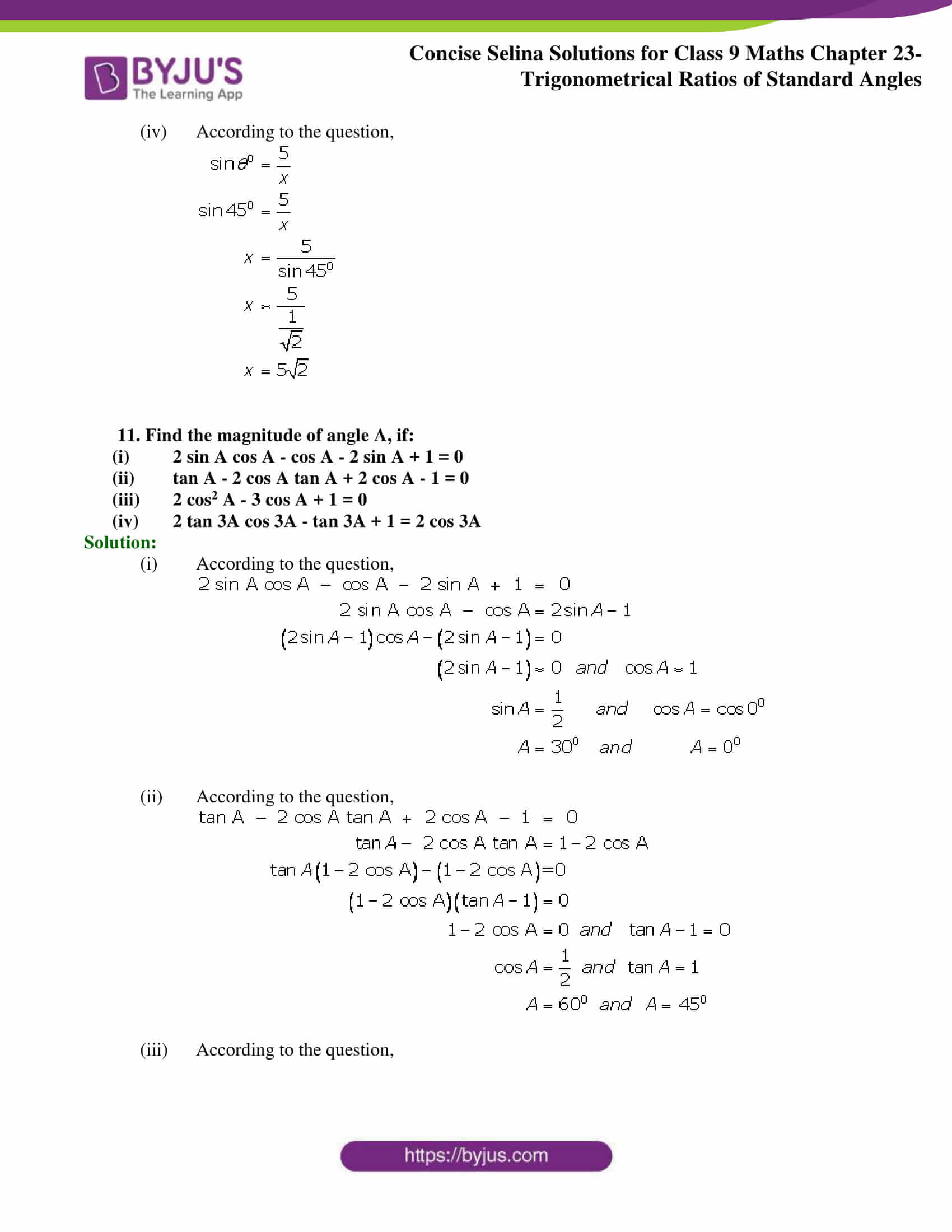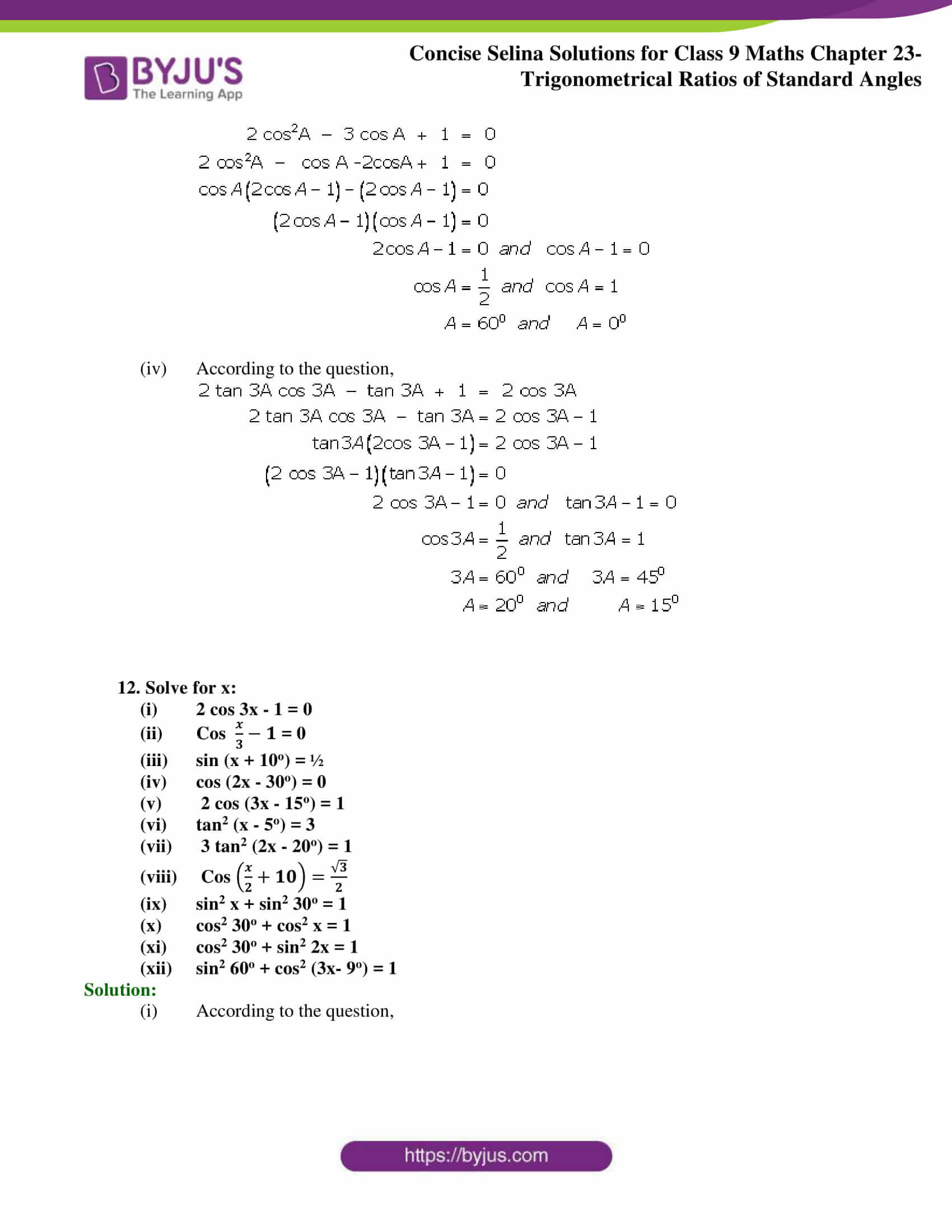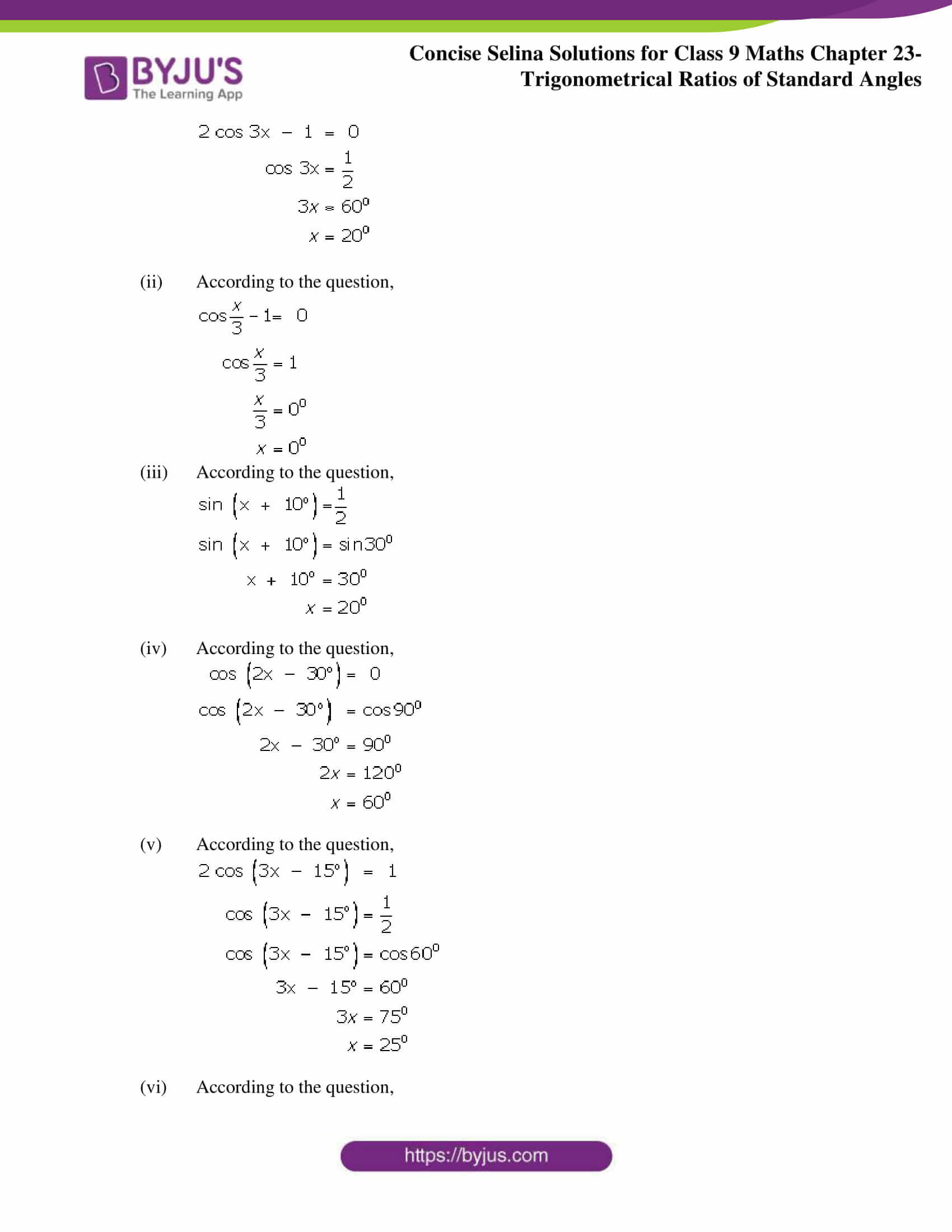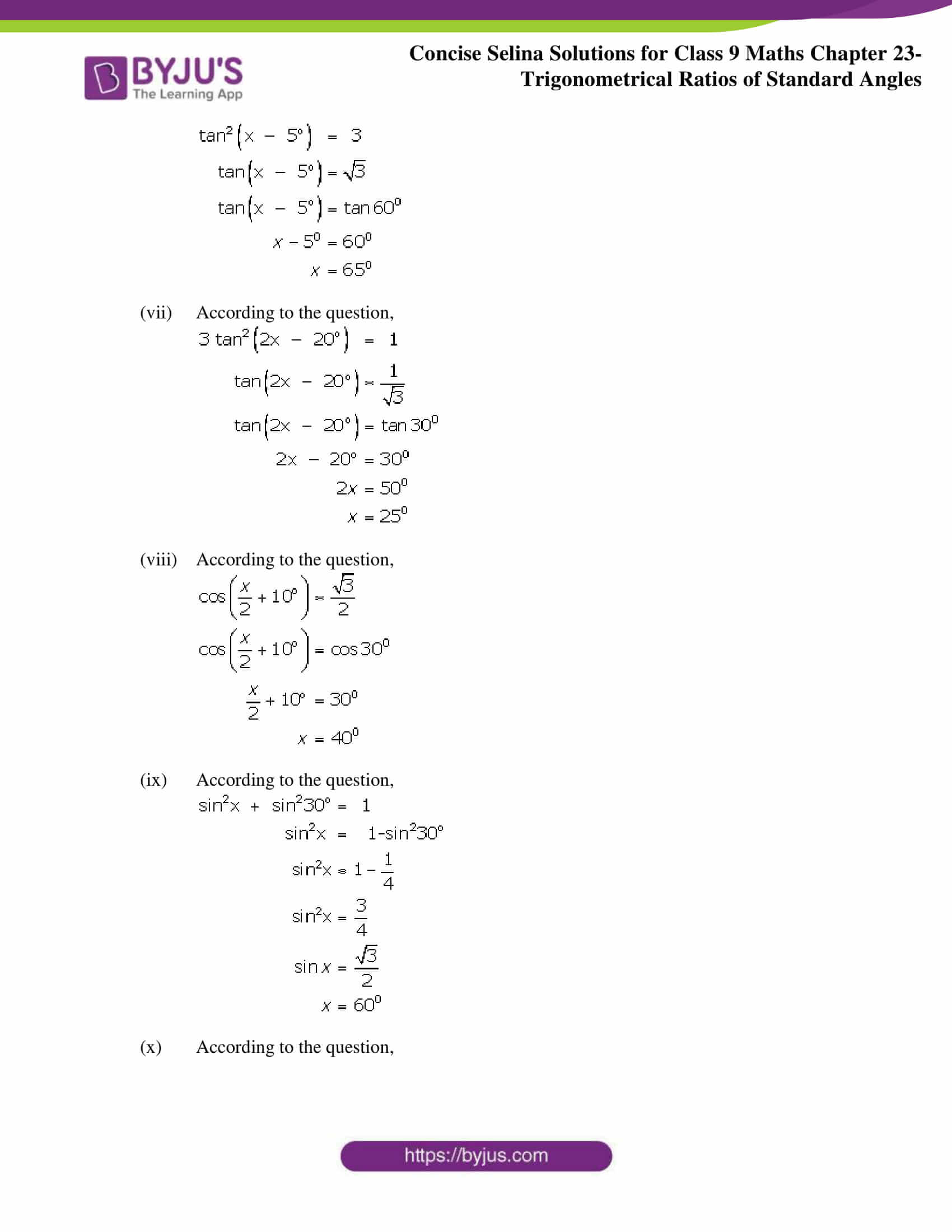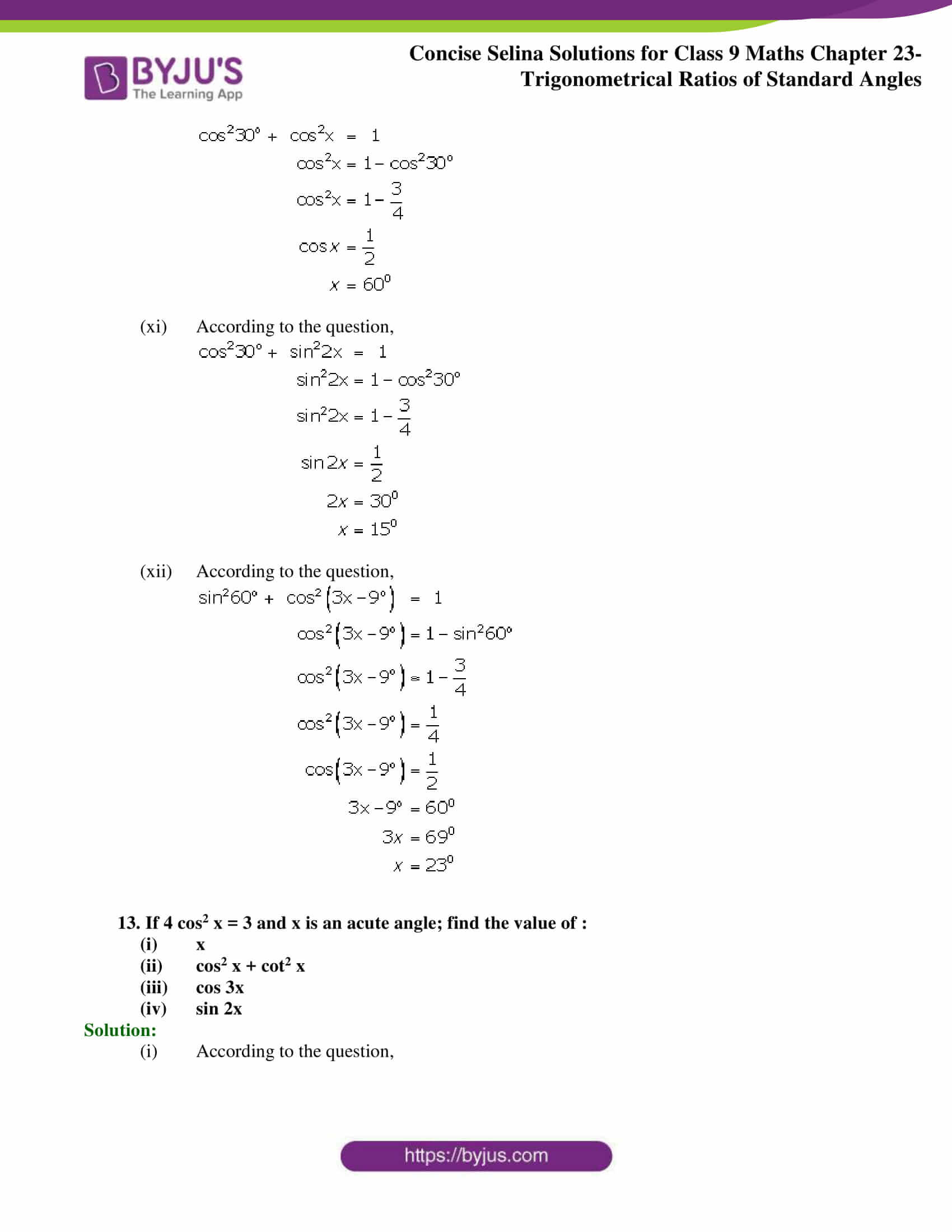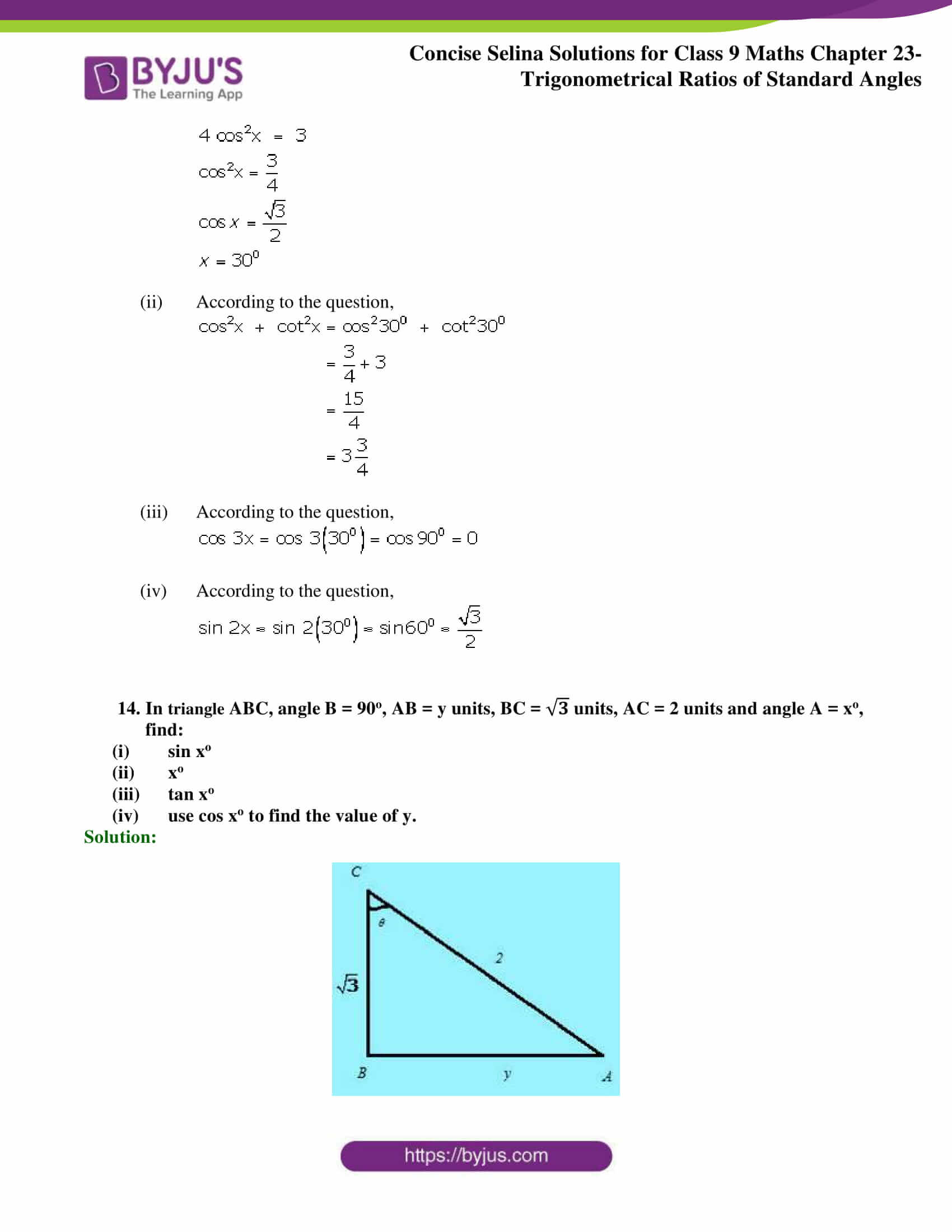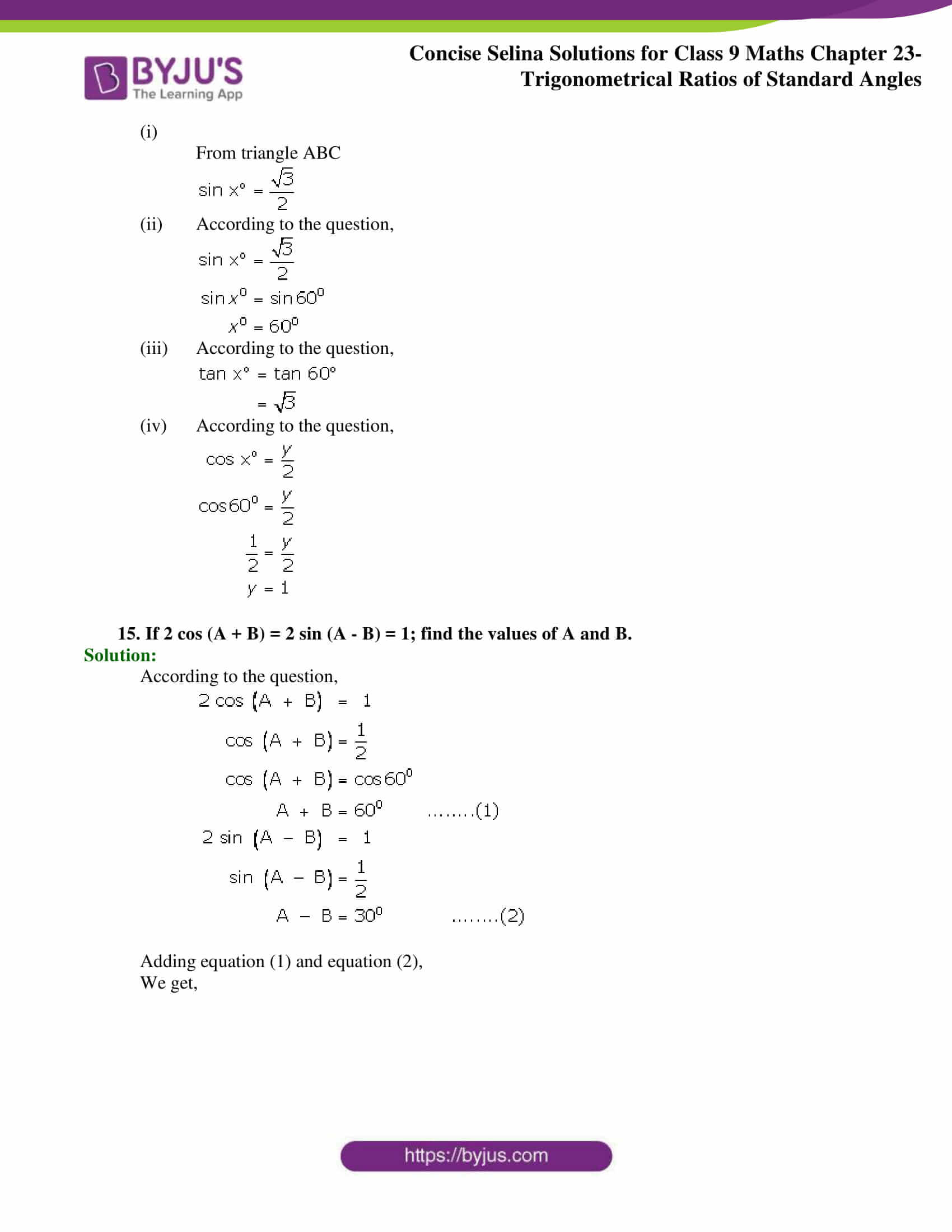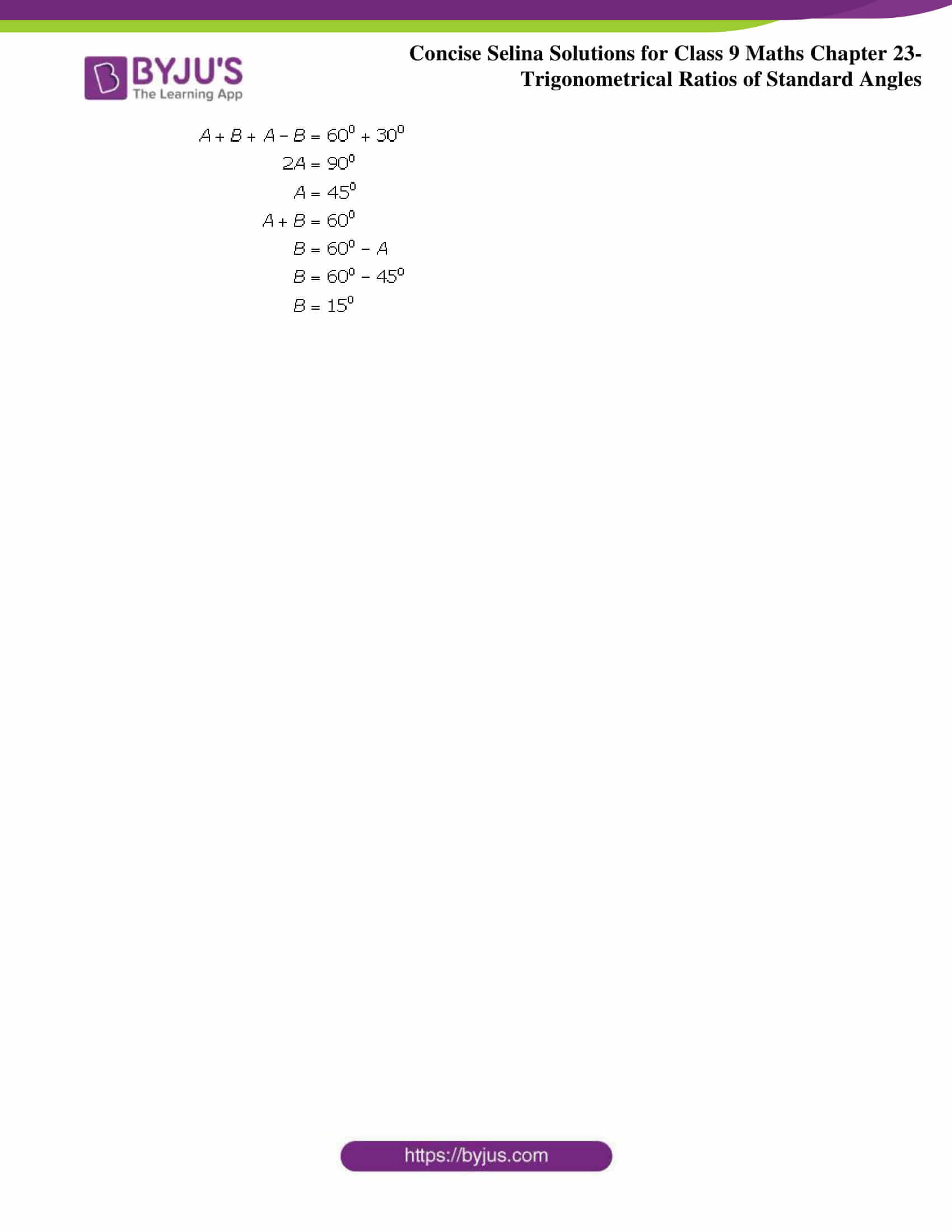## Selina Solutions for Class 9 Maths Chapter 23- Trigonometrical Ratios of Standard Angles

The Chapter 23, Trigonometrical Ratios of Standard Angles is composed of 3 exercises and the solutions given here contain answers to all the questions present in these exercises. Let us have a look at some of the topics that are being discussed in this chapter.

23.1 Trigonometrical Ratios of Angles 30o and 60o.

23.2 Trigonometrical Ratios of Angle 45o

23.3 Solving a trigonometric equation

## Selina Solutions for Class 9 Maths Chapter 23- Trigonometrical Ratios of Standard Angles

In Chapter 23 of Class 9, the students are taught about the Trigonometrical Ratios of Standard Angles. The chapter also includes the evaluation of an expression involving trigonometric ratios and problems related to it. Study Chapter 23 of Selina textbook to understand more about Trigonometrical Ratios of Standard Angles. Learn the Selina Solutions for Class 9 effectively to come out with flying colours in the examinations.